# Hsin-Po's WebsiteMath PhD @ UIUC

# Oriclip

One of my interests involves building binder clip sculptures. The name oriclip is inspired by origami, which stands for ori “fold” and kami “paper”. Note that binder clips are sometimes called foldover clip or foldback clip.

Fast scroll to

(The page is under construction; check back periodically.)

## Special case

### 2-clip constructions

#### 2-ftf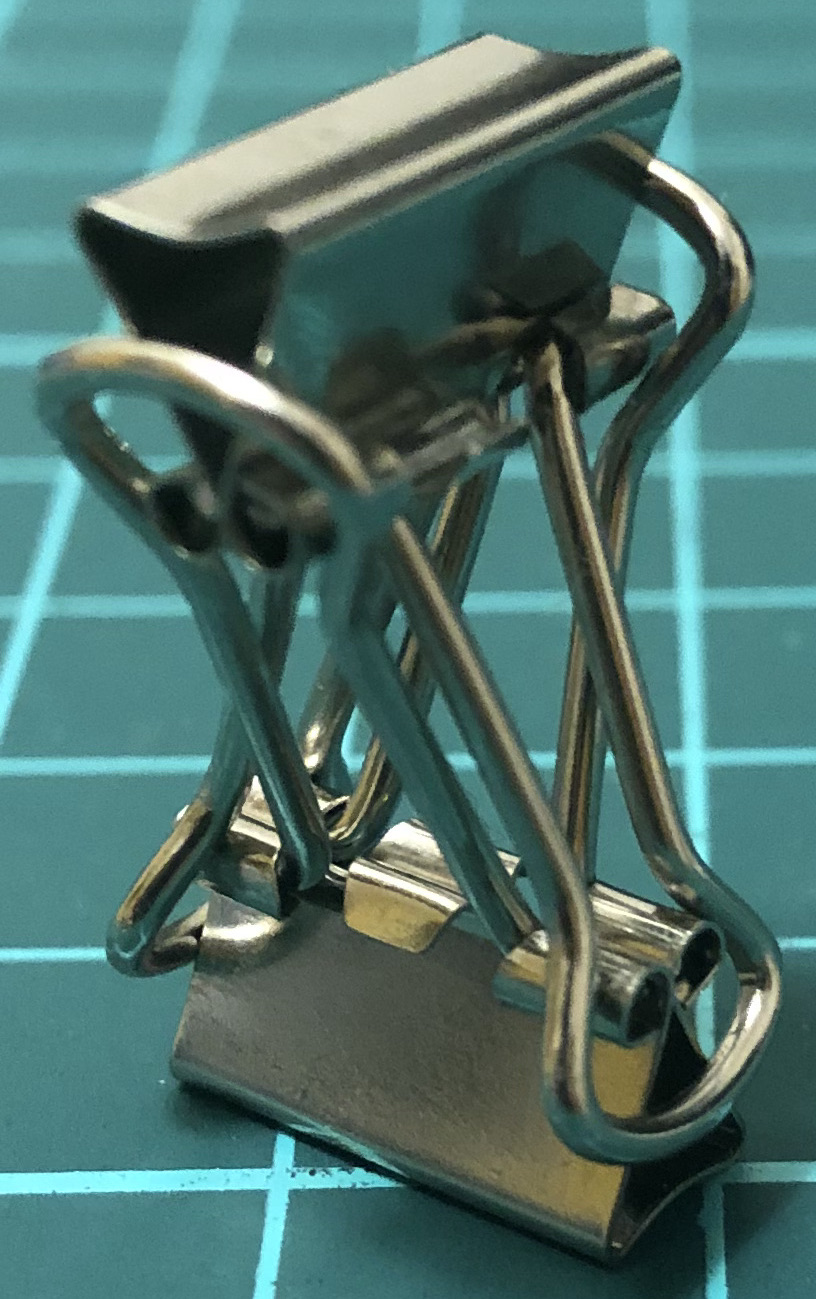↑ # Clips = 2

#### 2-btb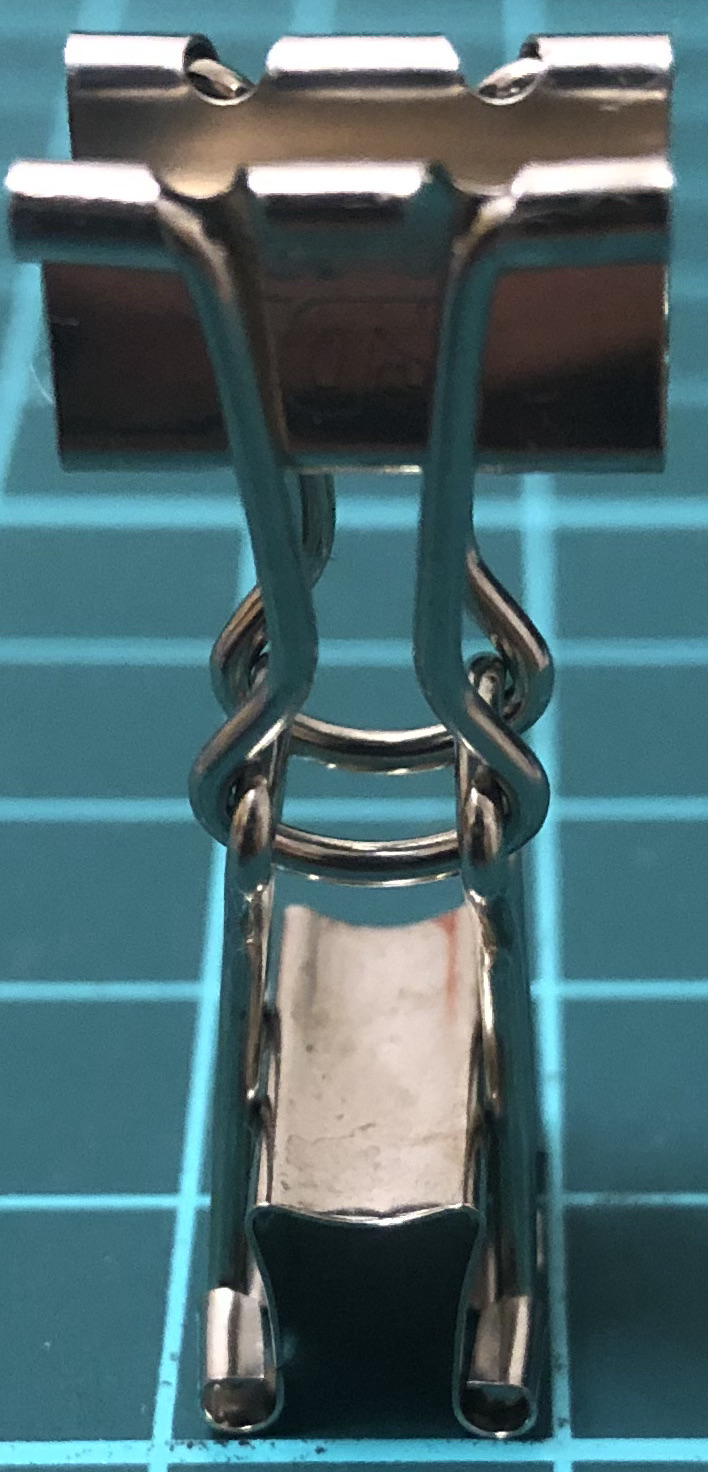↑ # Clips = 2

### 6-clip constructions

#### 6-cycle↑ # Clips = 6
↑ Base = triangular antiprism
↑ Symmetry = triangular antiprism’s rotations = $D_6$ of order 6

#### 6-dense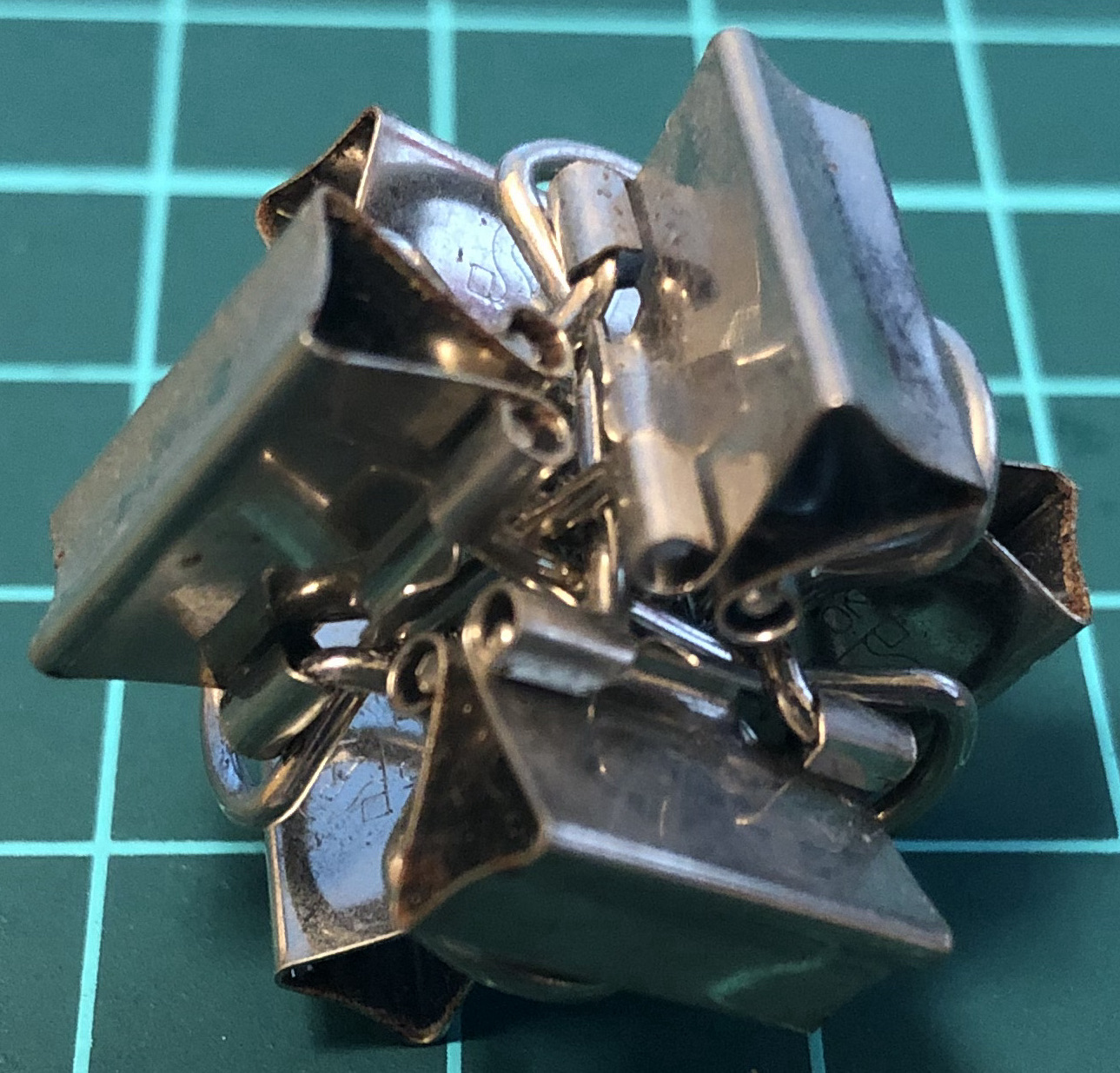↑ # Clips = 6
↑ Base = six-piece burr
↑ Symmetry = triangular antiprism’s rotations = $D_6$ of order 6

#### 6-wedge↑ # Clips = 6
↑ Base = six-piece burr
↑ Symmetry = tetrahedron’s rotations = $A_4$ of order 12

#### 6-fitin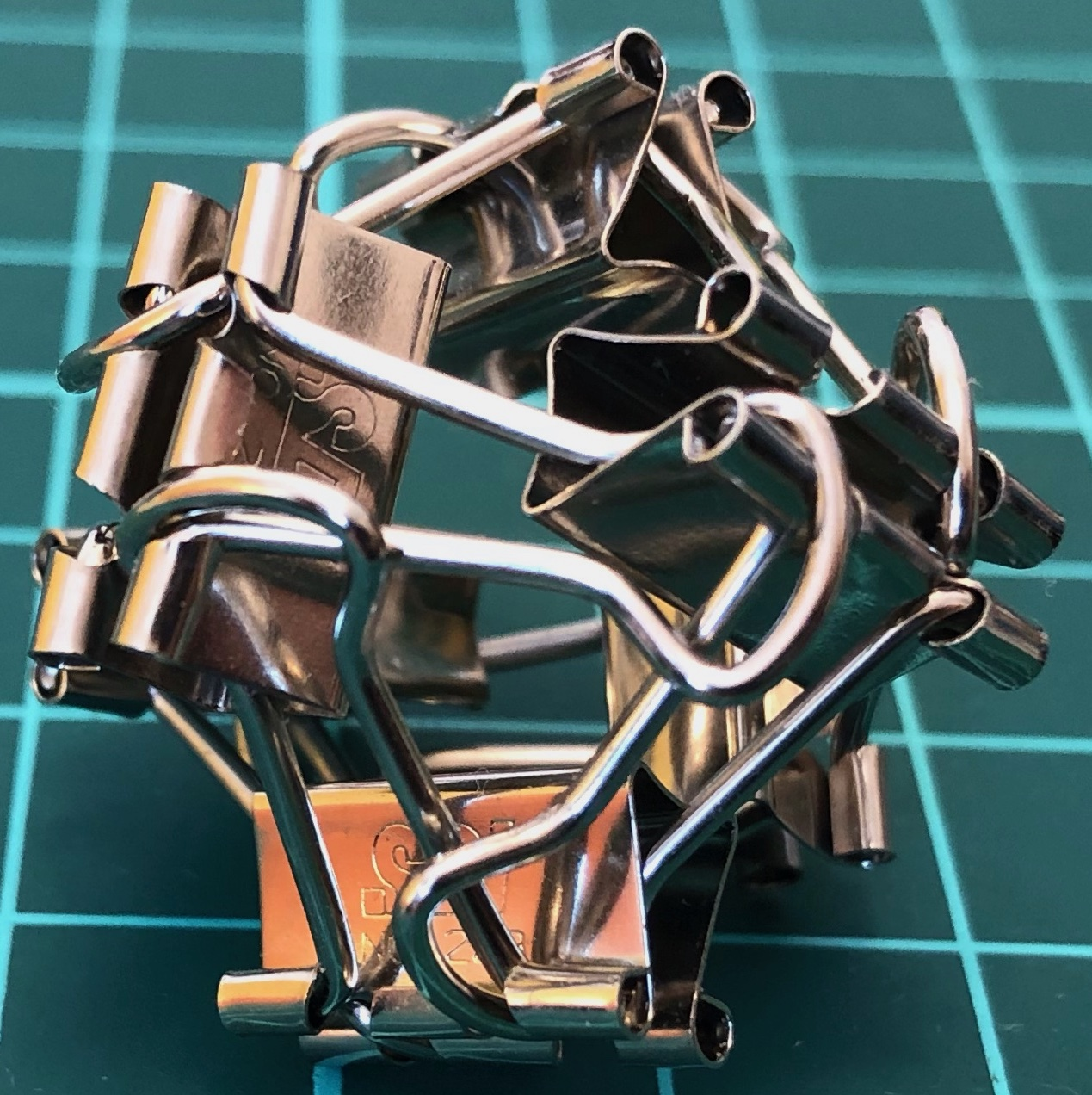↑ # Clips = 6
↑ Base = octahedron
↑ Symmetry = tetrahedron’s rotations = $A_4$ of order 12

#### 6-twist↑ # Clips = 6
↑ Base = octahedron
↑ Symmetry = tetrahedron’s rotations = $A_4$ of order 12

#### 6-cross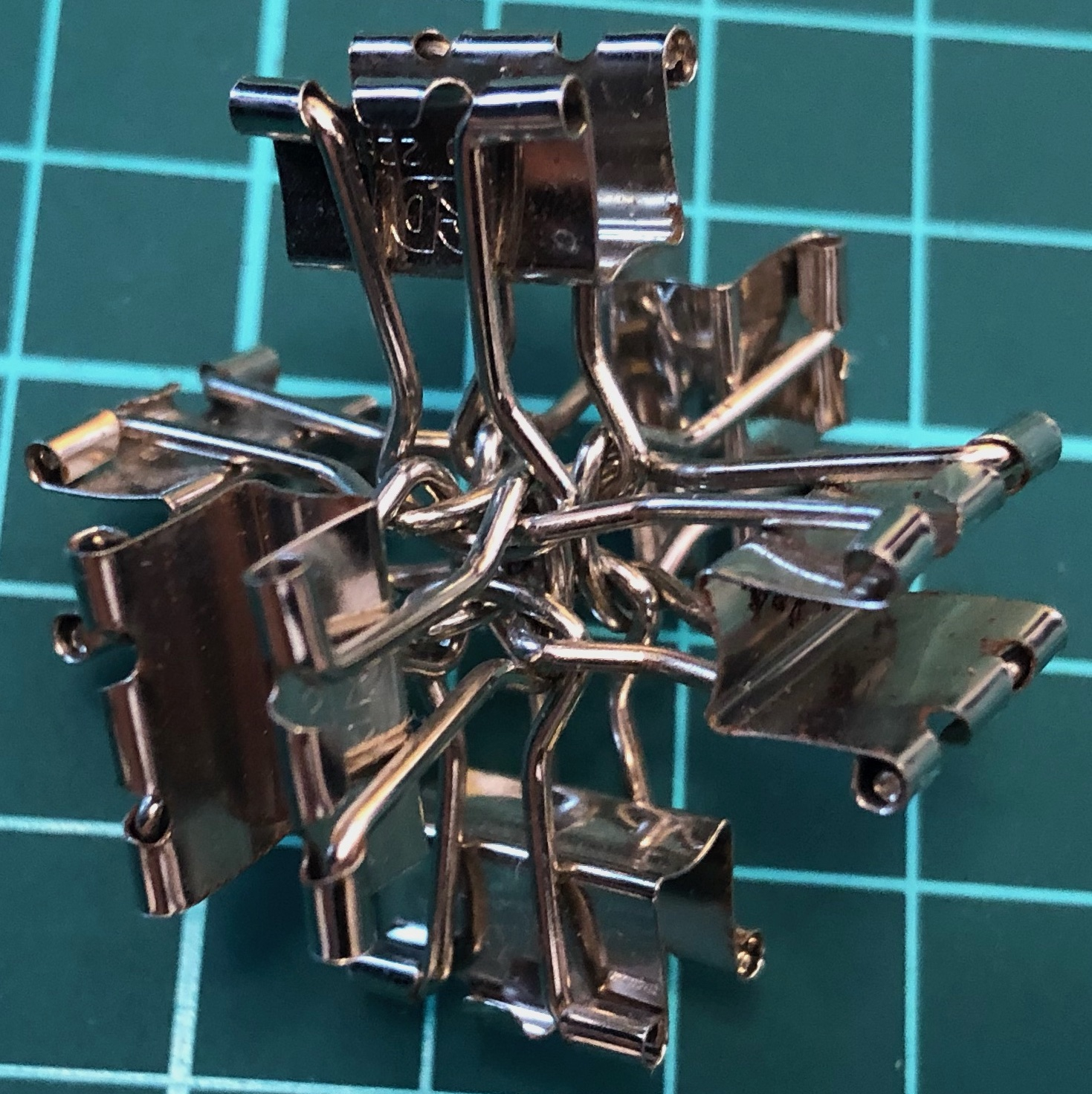↑ # Clips = 6
↑ Base = three-piece burr
↑ Symmetry = pyritohedron’s rotations and reflections = $A_4\times C_2$ of order 24

#### 6-spike↑ # Clips = 6
↑ Base = six-piece burr
↑ Symmetry = pyritohedron’s rotations and reflections = $A_4\times C_2$ of order 24

#### 6-stand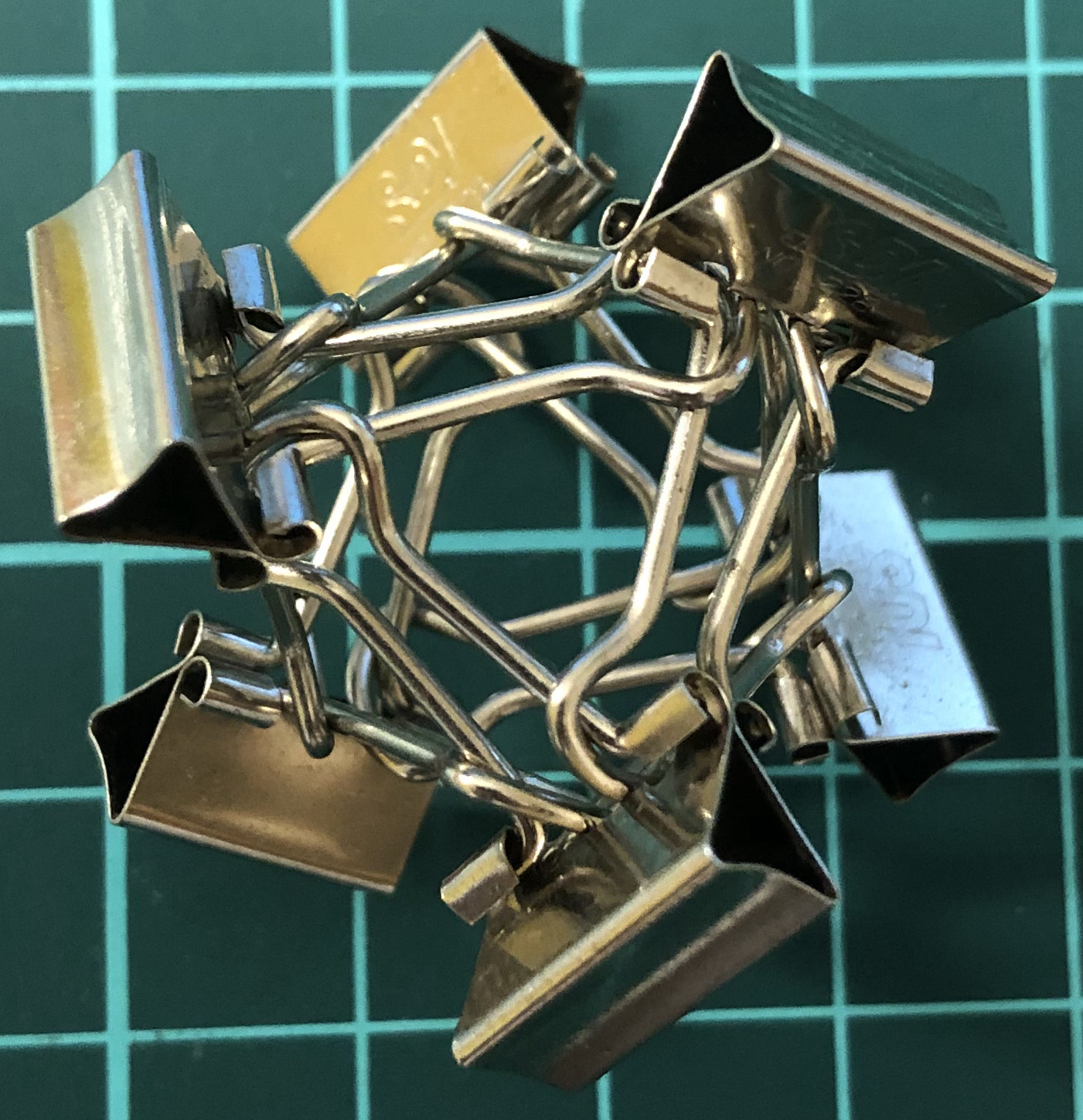↑ # Clips = 6
↑ Base = octahedron
↑ Symmetry = pyritohedron’s rotations and reflections = $A_4\times C_2$ of order 24

#### Q12-aC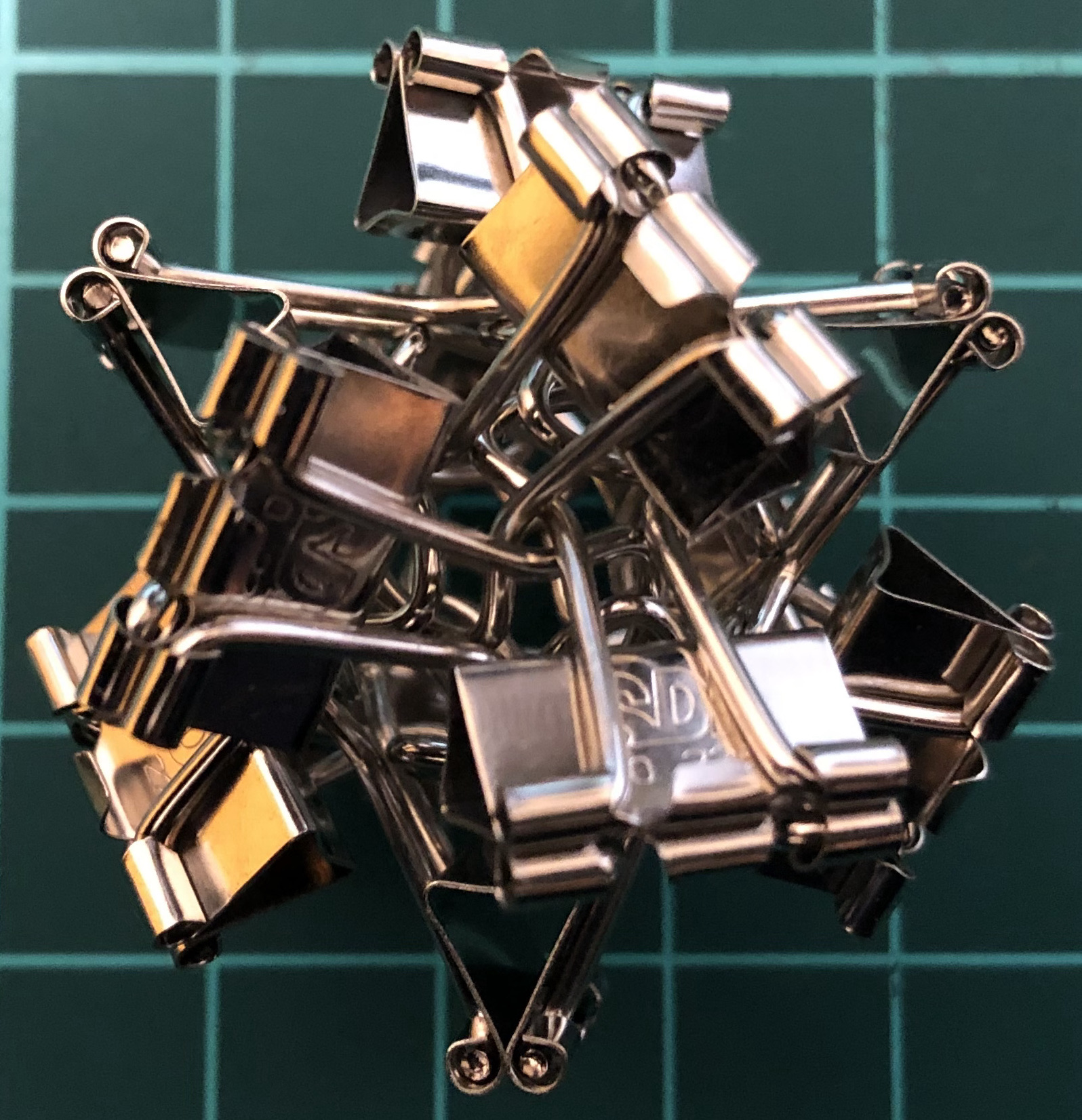↑ # Clips = 12
↑ Base = cuboctahedron

### S-series

One clip = one vertex. One handle = one edge.

#### S12-aC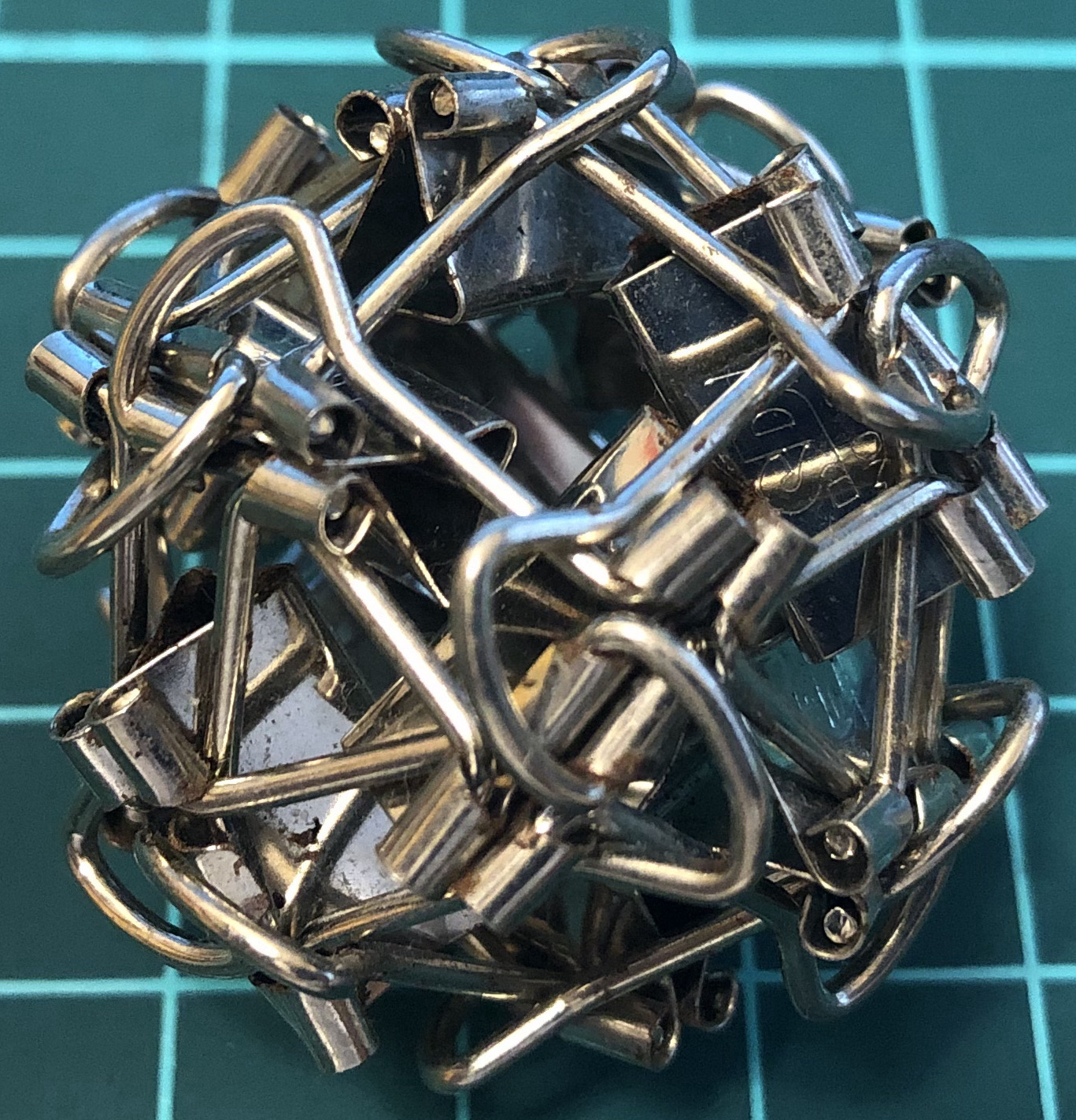↑ # Clips = 12
↑ Base = cuboctahedron
↑ Symmetry = cube’s rotations = $S_4$ of order 24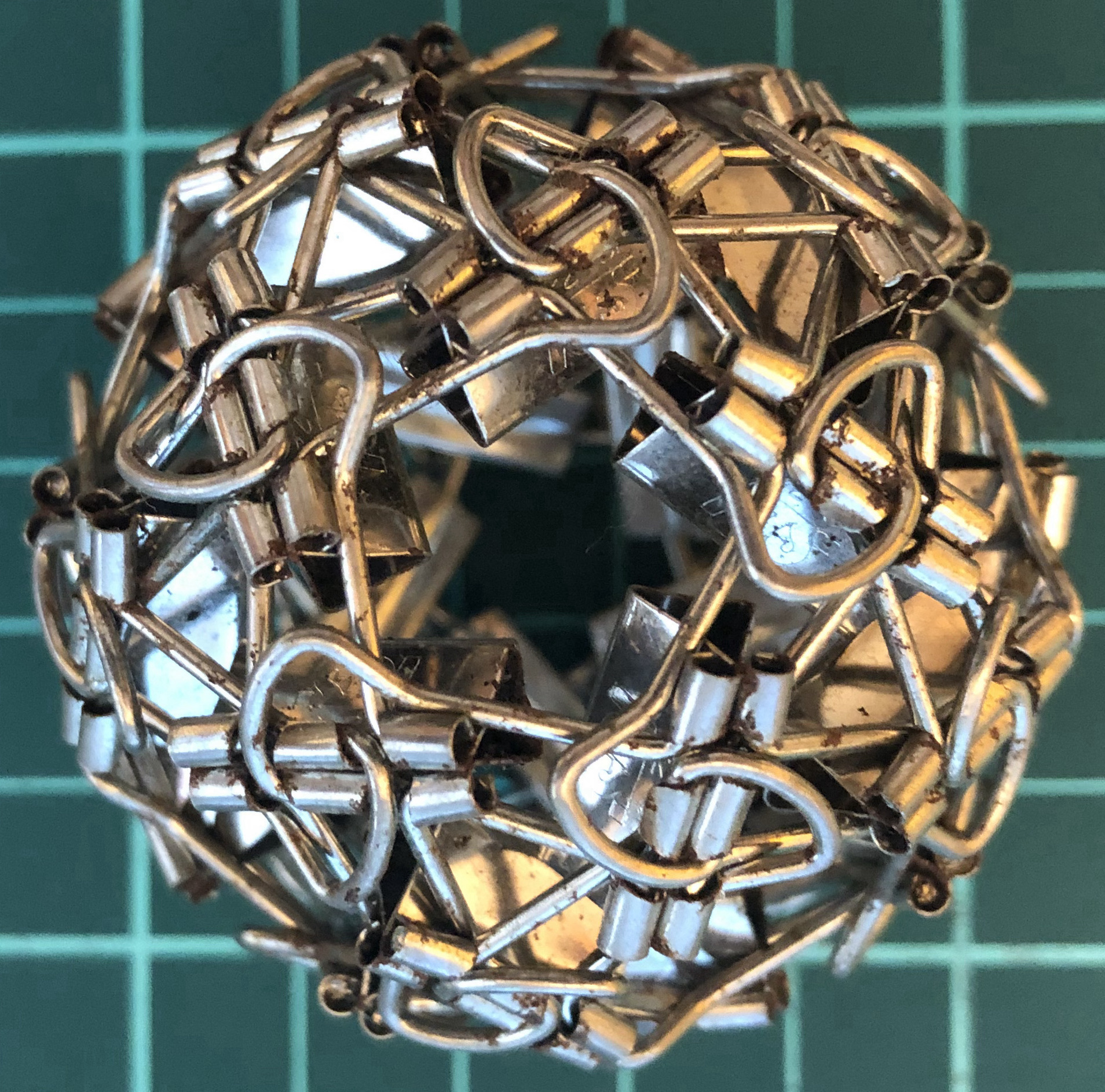↑ # Clips = 30
↑ Base = icosidodecahedron
↑ Symmetry = dodecahedron’s rotations = $A_5$ of order 60

### A-series

One clip = one edge.

#### A12-O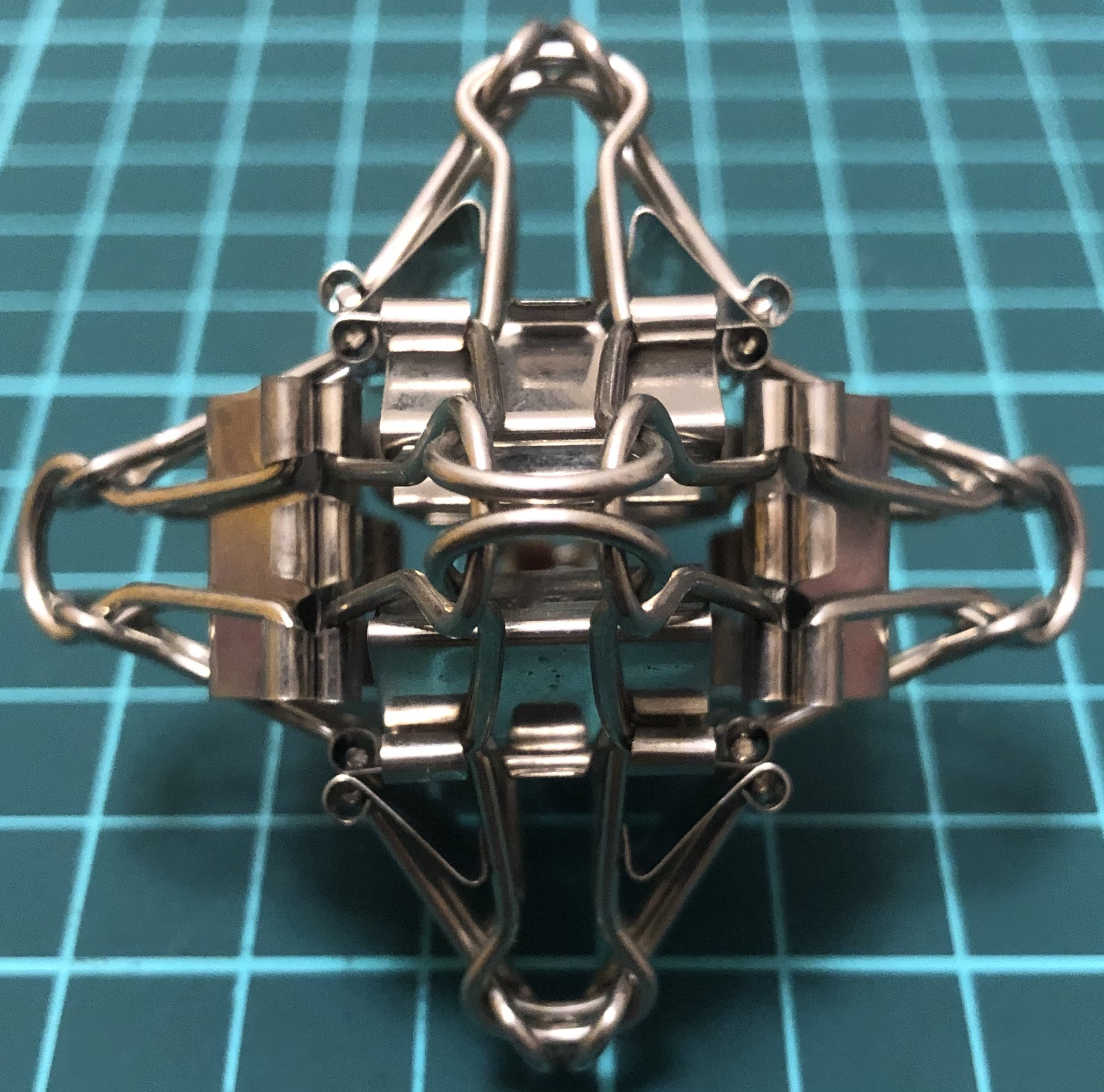↑ # Clips = 12
↑ Base = octahedron
↑ Symmetry = pyritohedron’s rotations and reflections = $A_4\times C_2$ of order 24

#### A24-aC↑ # Clips = 24
↑ Base = cuboctahedron
↑ Symmetry = cube’s rotations = $S_4$ of order 24

#### A36-kC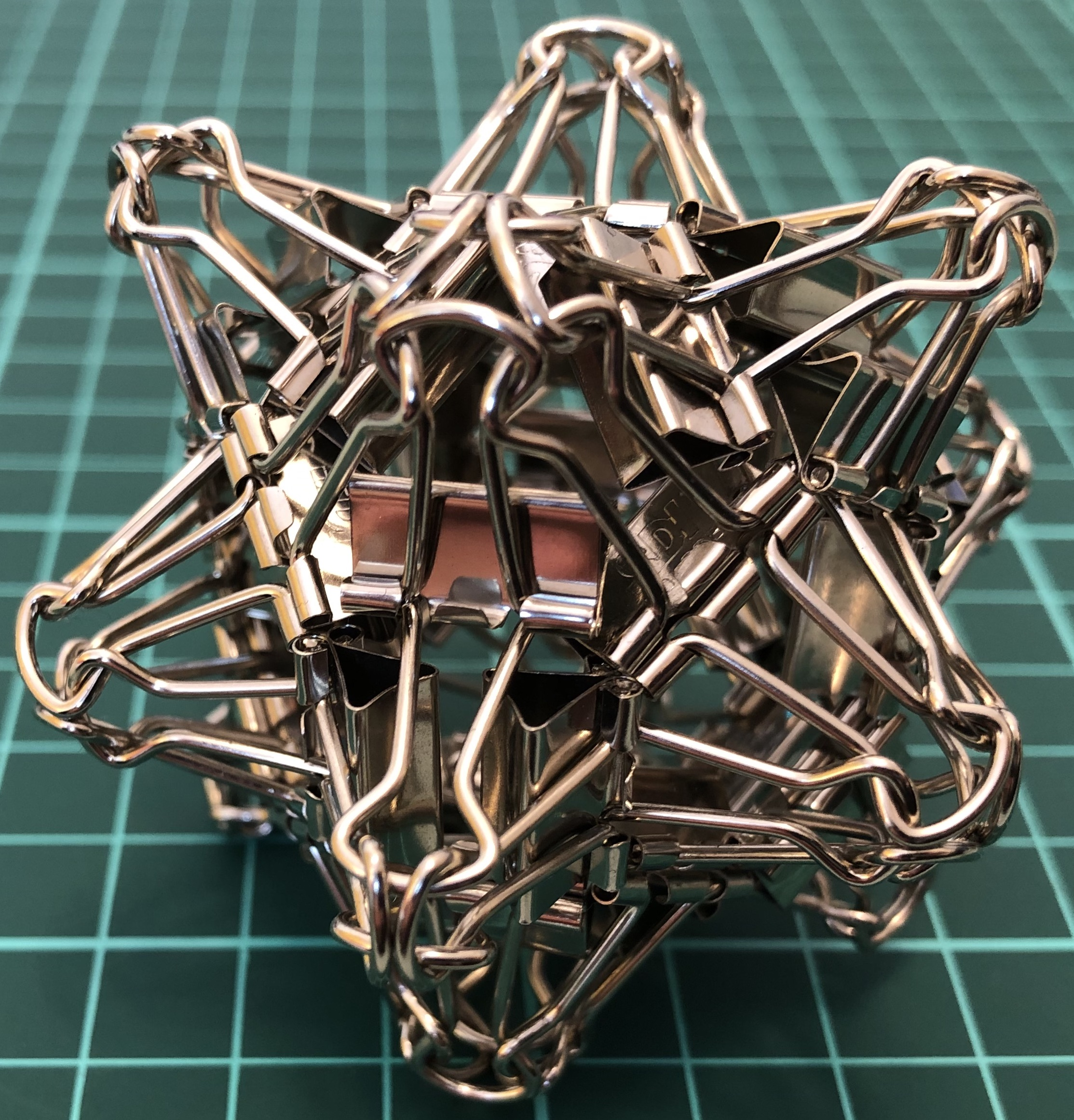↑ # Clips = 36
↑ Base = tetrakis hexahedron
↑ Symmetry = pyritohedron’s rotations and reflections = $A_4\times C_2$ of order 24

#### A48-aaC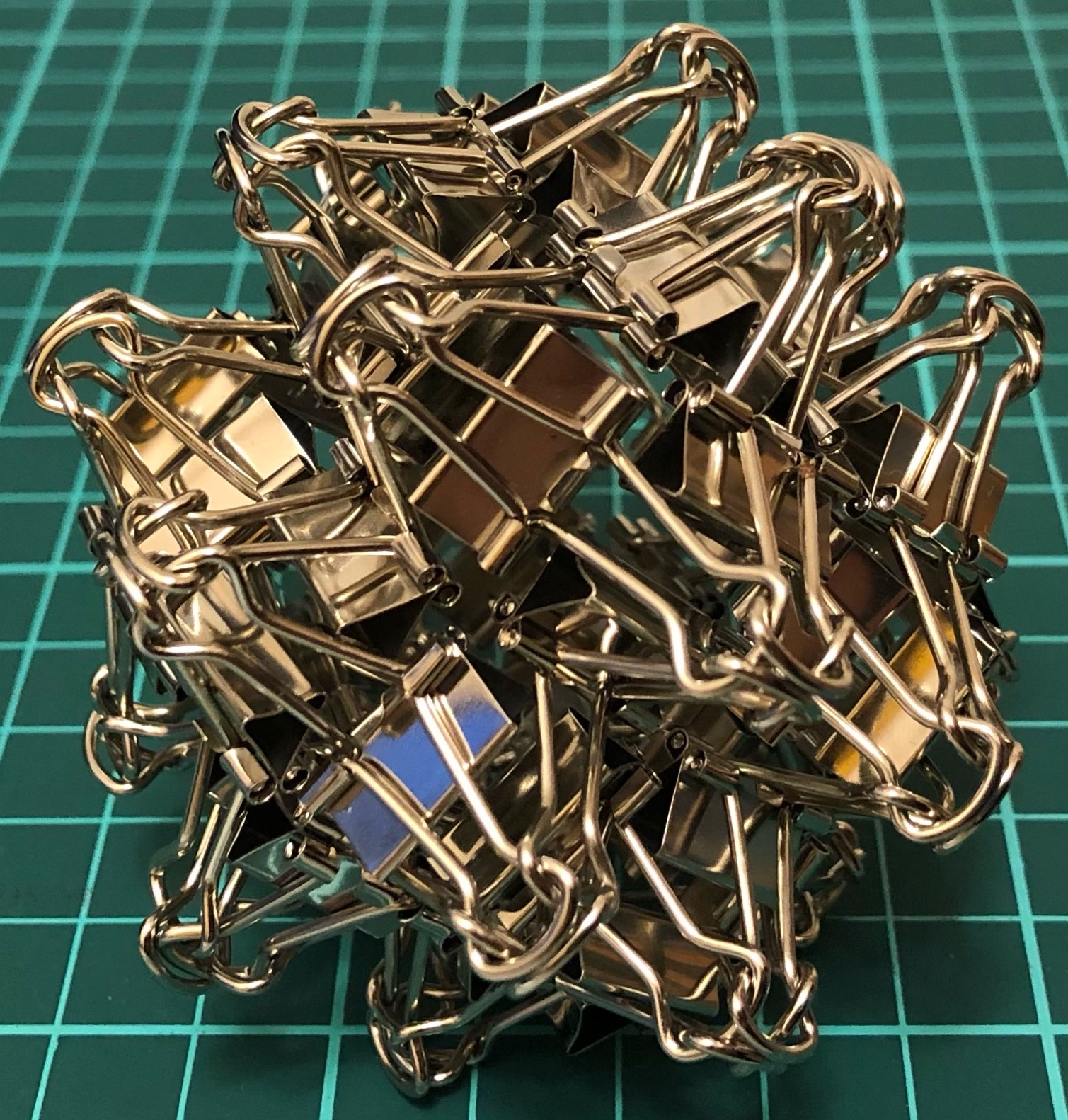↑ # Clips = 48
↑ Base = rhombicuboctahedron
↑ Symmetry = cube’s rotations = $S_4$ of order 24

## Vertex unit

### Φ-series

Three clips = one Φ-vertex = one vertex.

#### Φ24-C↑ # Clips = 24
↑ Base = cube
↑ Vertex config = 4.4.4
↑ Symmetry = cube’s rotations = $S_4$ of order 24
↑ Dual = Φ24-O

#### Φ24-O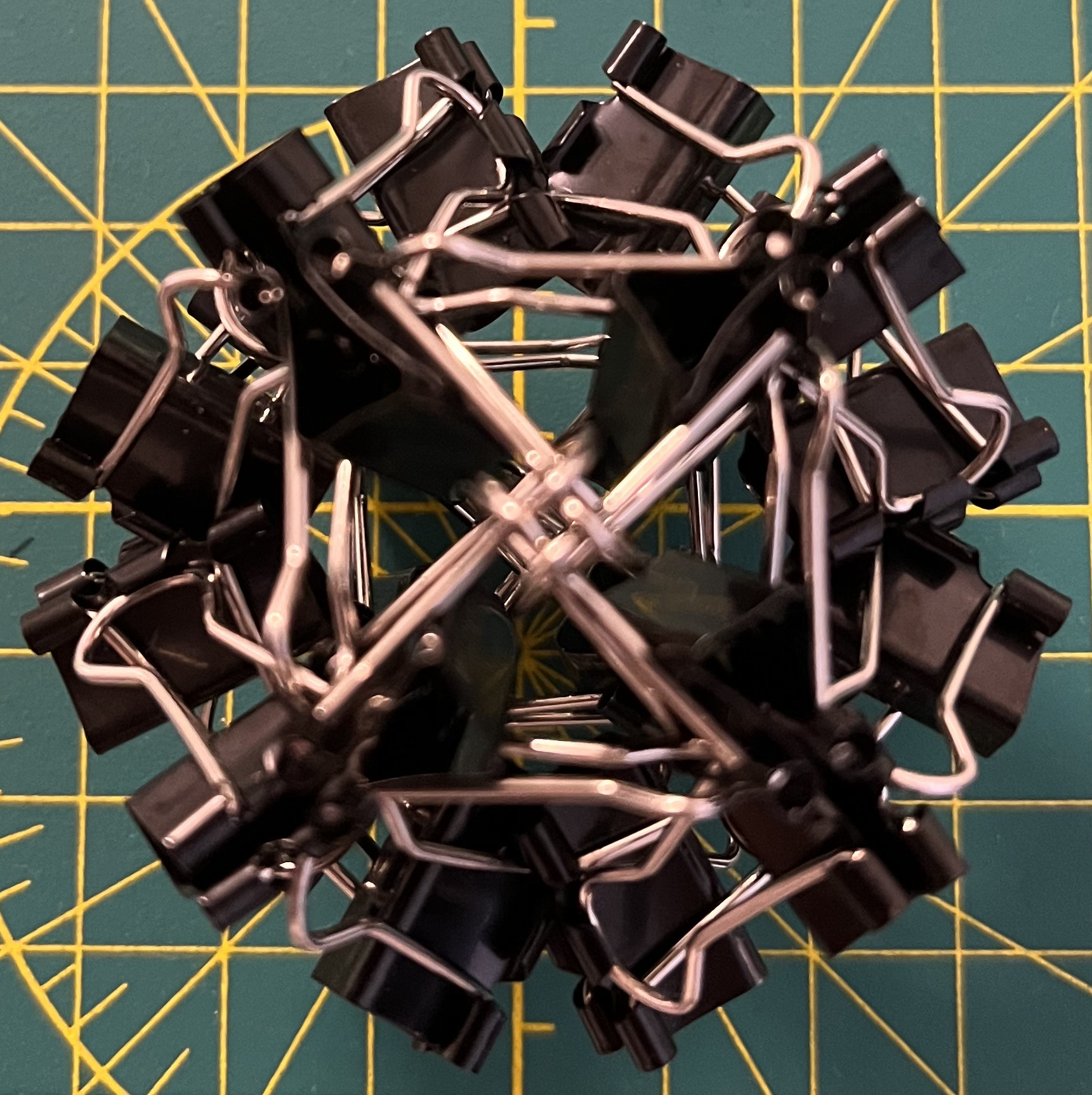↑ # Clips = 24
↑ Base = octahedron
↑ Vertex config = 3.3.3.3
↑ Symmetry = cube’s rotations = $S_4$ of order 24
↑ Dual = Φ24-C

### Δ-series

Three clips = one Δ-vertex = one vertex.

#### Δ60-D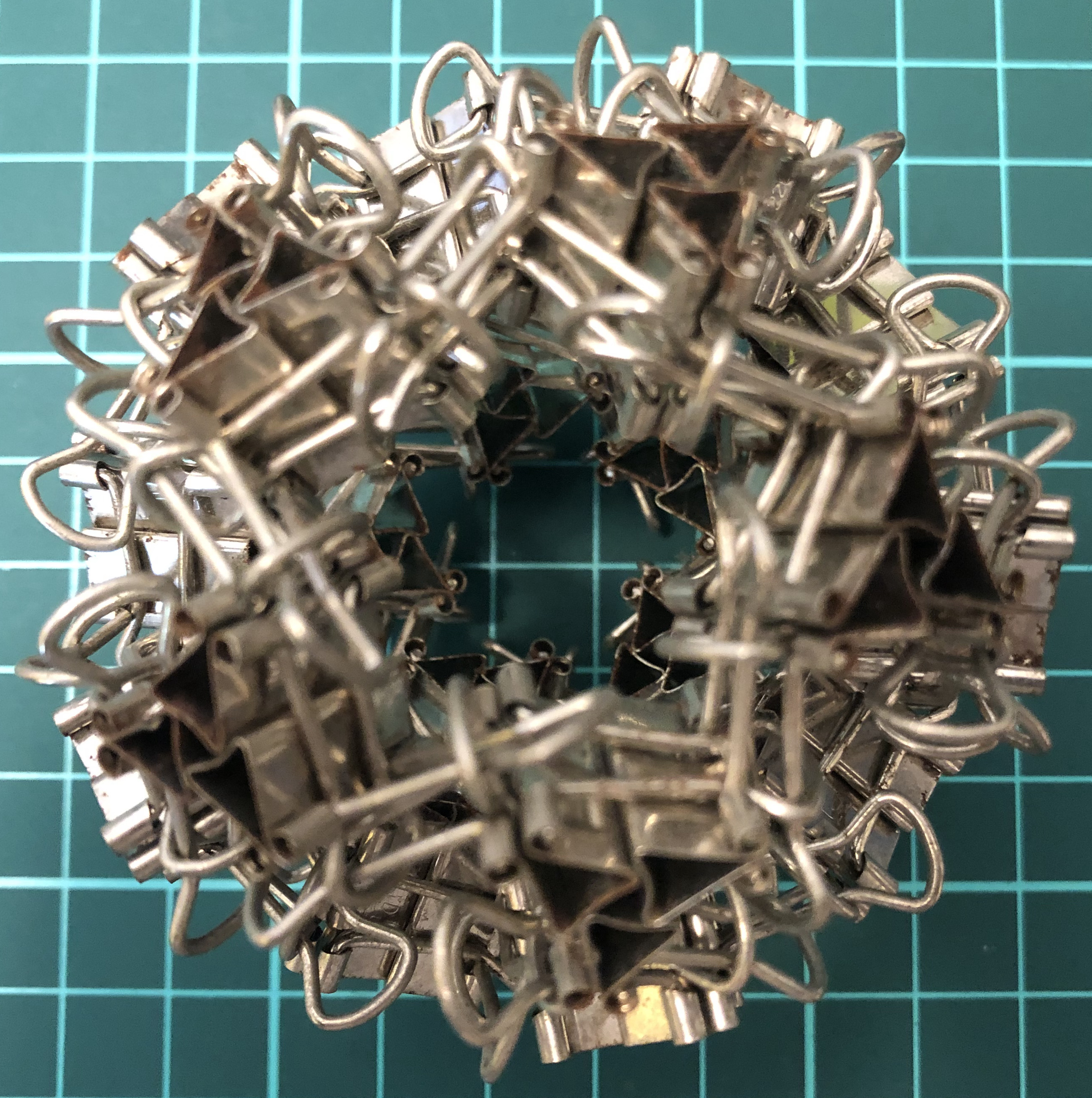↑ # Clips = 60
↑ Vertex config = 5.5.5
↑ Base = dodecahedron
↑ Symmetry = dodecahedron’s rotations = $A_5$ of order 60

#### Δ180-tI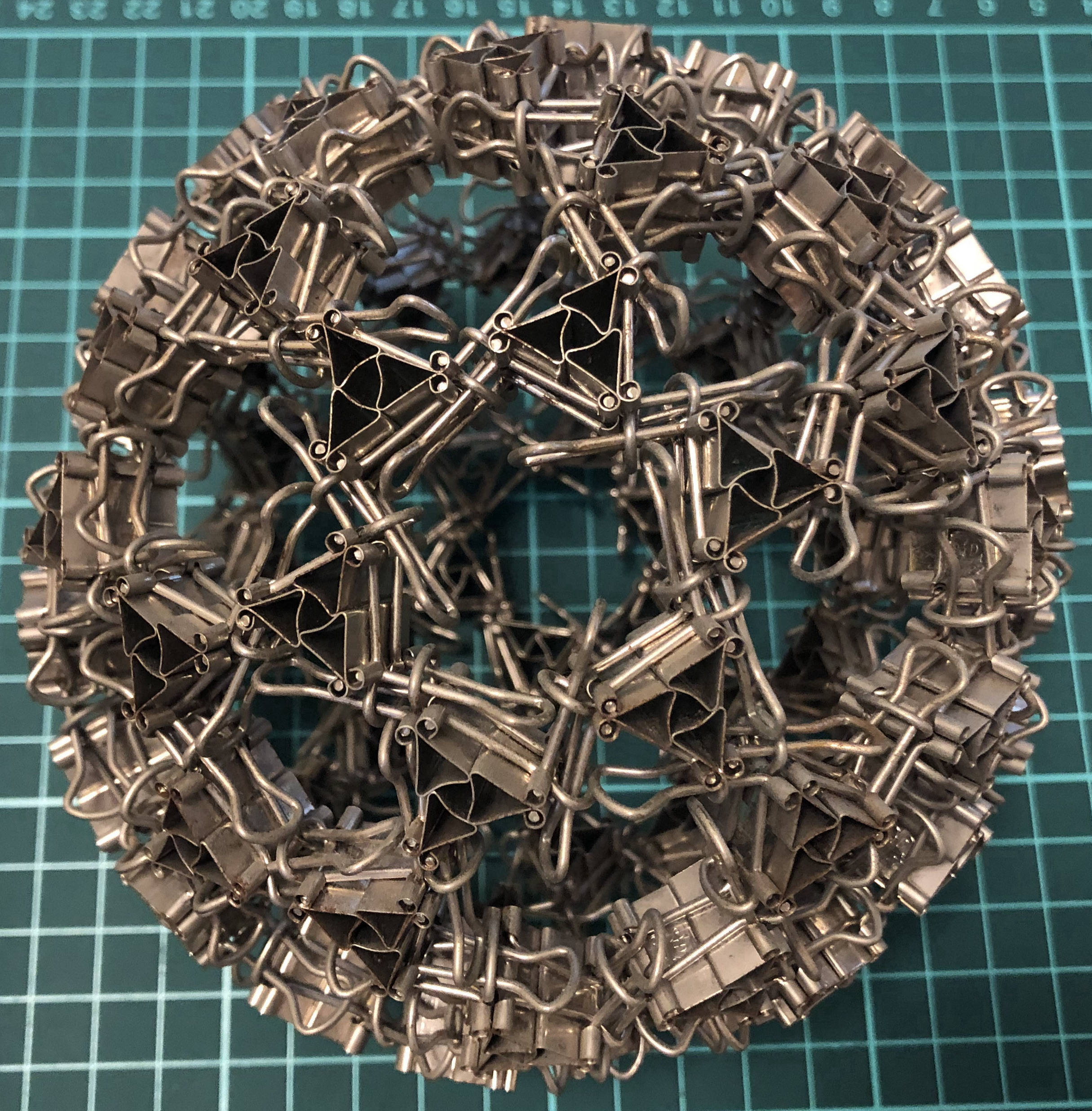↑ # Clips = 180
↑ Vertex config = 5.6.6
↑ Base = truncated icosahedron
↑ Symmetry = dodecahedron’s rotations = $A_5$ of order 60

## Edge unit

### X-series

Two clips = one X-edge = one edge.

#### X12-T↑ # Clips = 12
↑ Base = tetrahedron
↑ Vertex config = 3.3.3
↑ Symmetry = tetrahedron’s rotations = $A_4$ of order 12
↑ Dual = itself

#### X24-C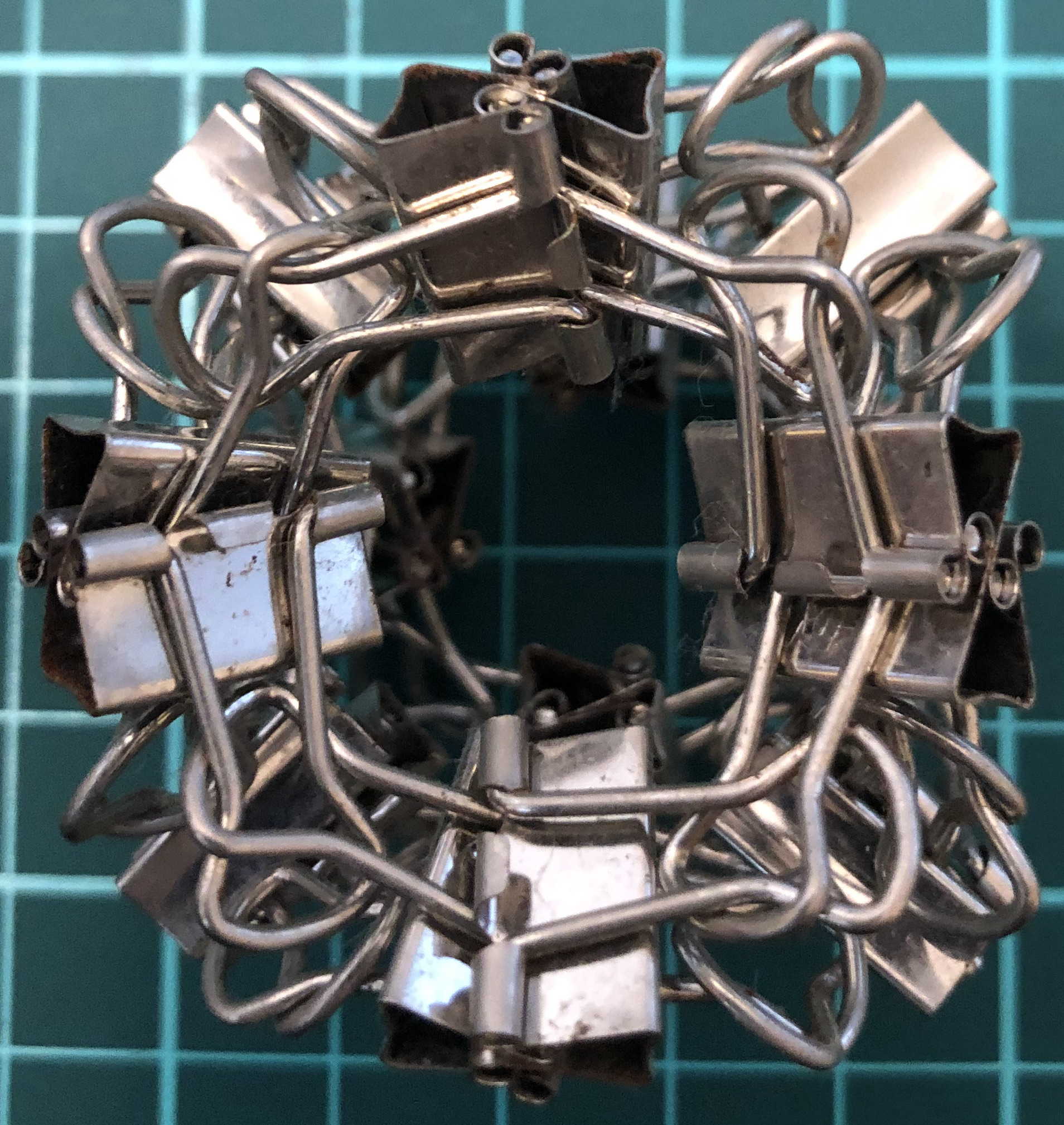↑ # Clips = 24
↑ base = cube
↑ Vertex config = 4.4.4
↑ Symmetry = cube’s rotations = $S_4$ of order 24
↑ dual = X24-O

#### X24-O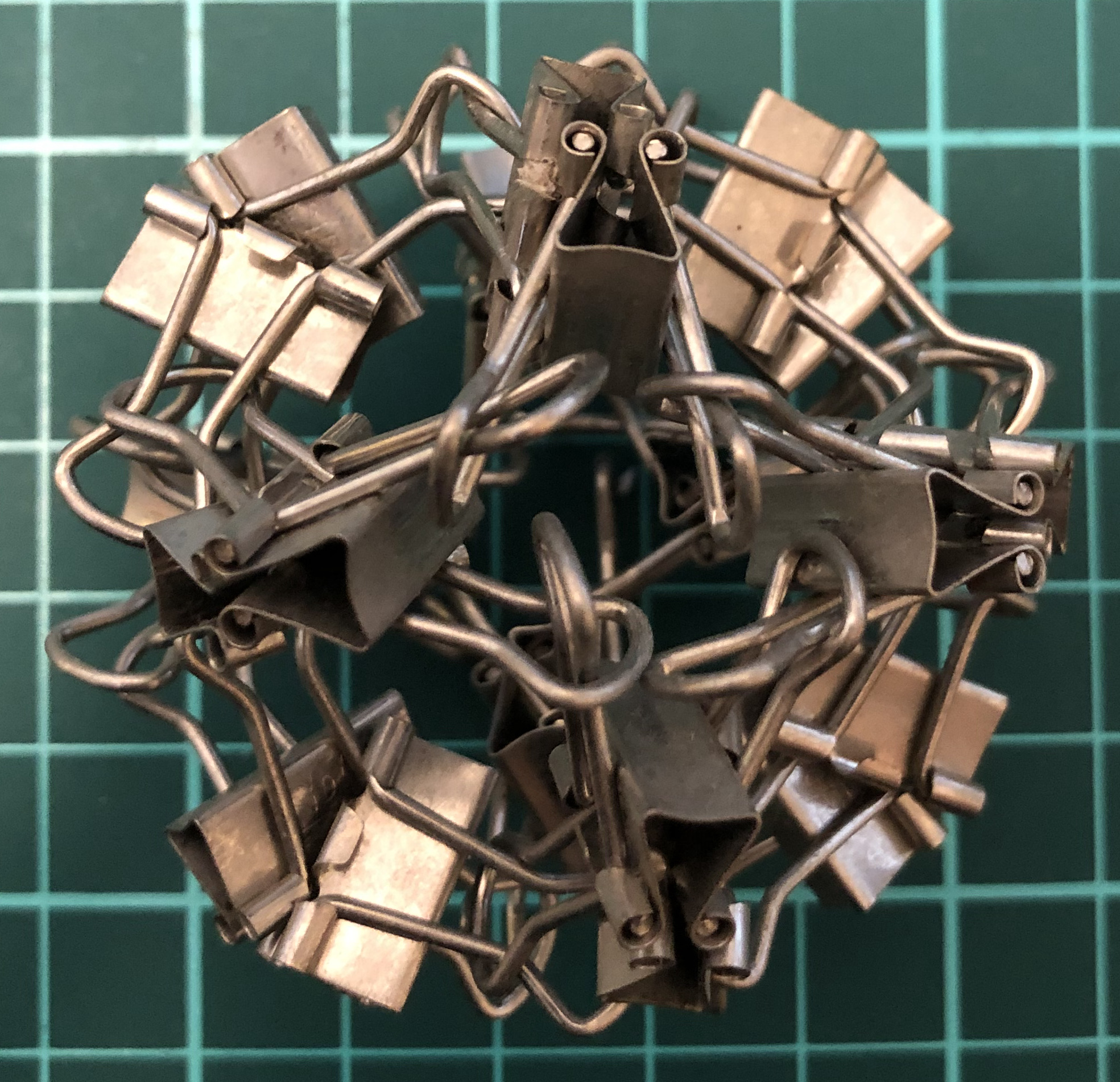↑ # Clips = 24
↑ base = octahedron
↑ Vertex config = 3.3.3.3
↑ Symmetry = cube’s rotations = $S_4$ of order 24
↑ dual = X24-C

#### X60-D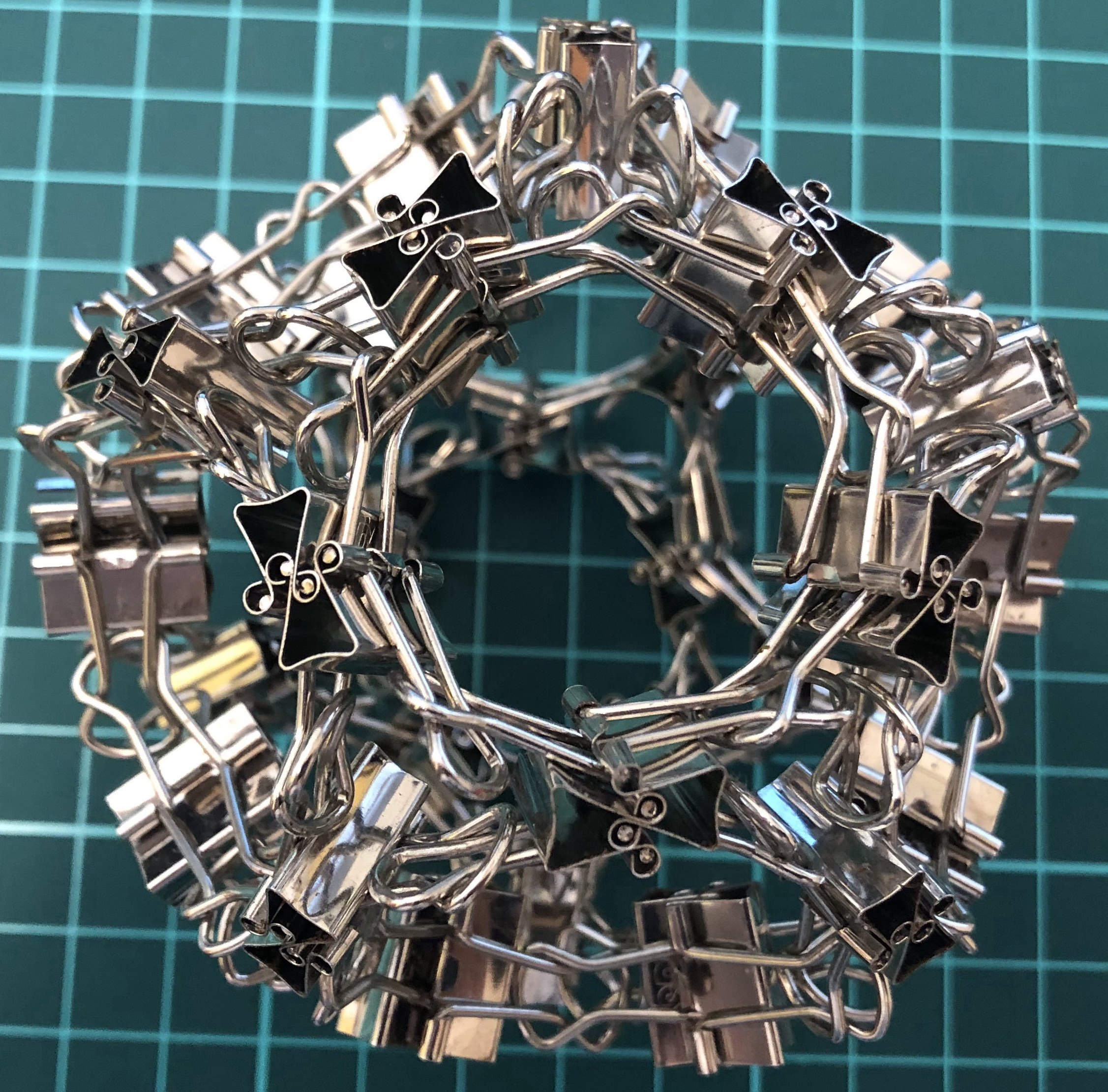↑ # Clips = 30
↑ Vertex config = 5.5.5
↑ Base = dodecahedron
↑ Symmetry = dodecahedron’s rotations = $A_5$ of order 60
↑ dual = X60-I

#### X60-I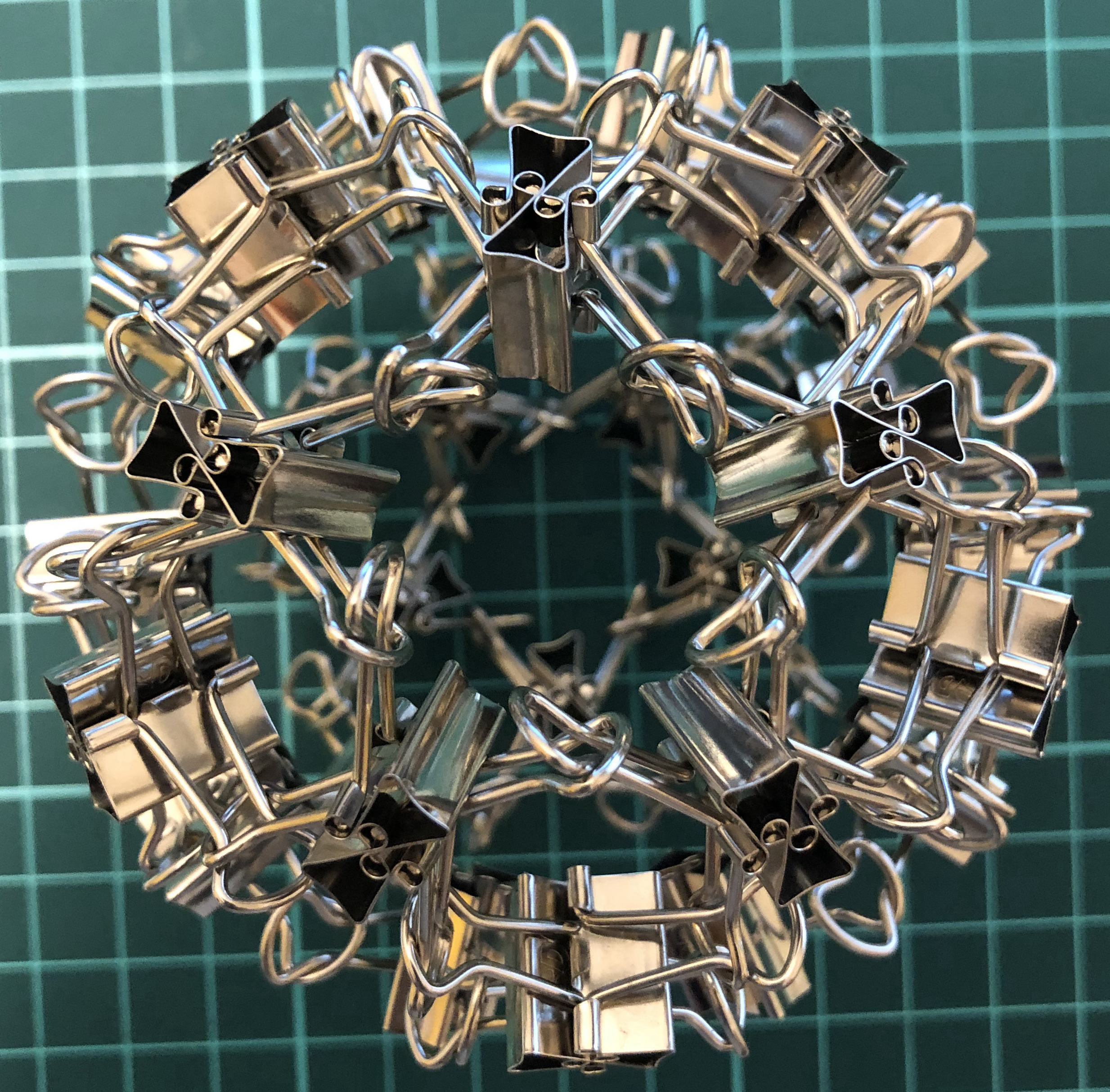↑ # Clips = 30
↑ Vertex config = 3.3.3.3.3
↑ Base = icosahedron
↑ Symmetry = dodecahedron’s rotations = $A_5$ of order 60
↑ dual = X60-D

### L-series

Two clips = one L-edge = one edge.

#### L12-T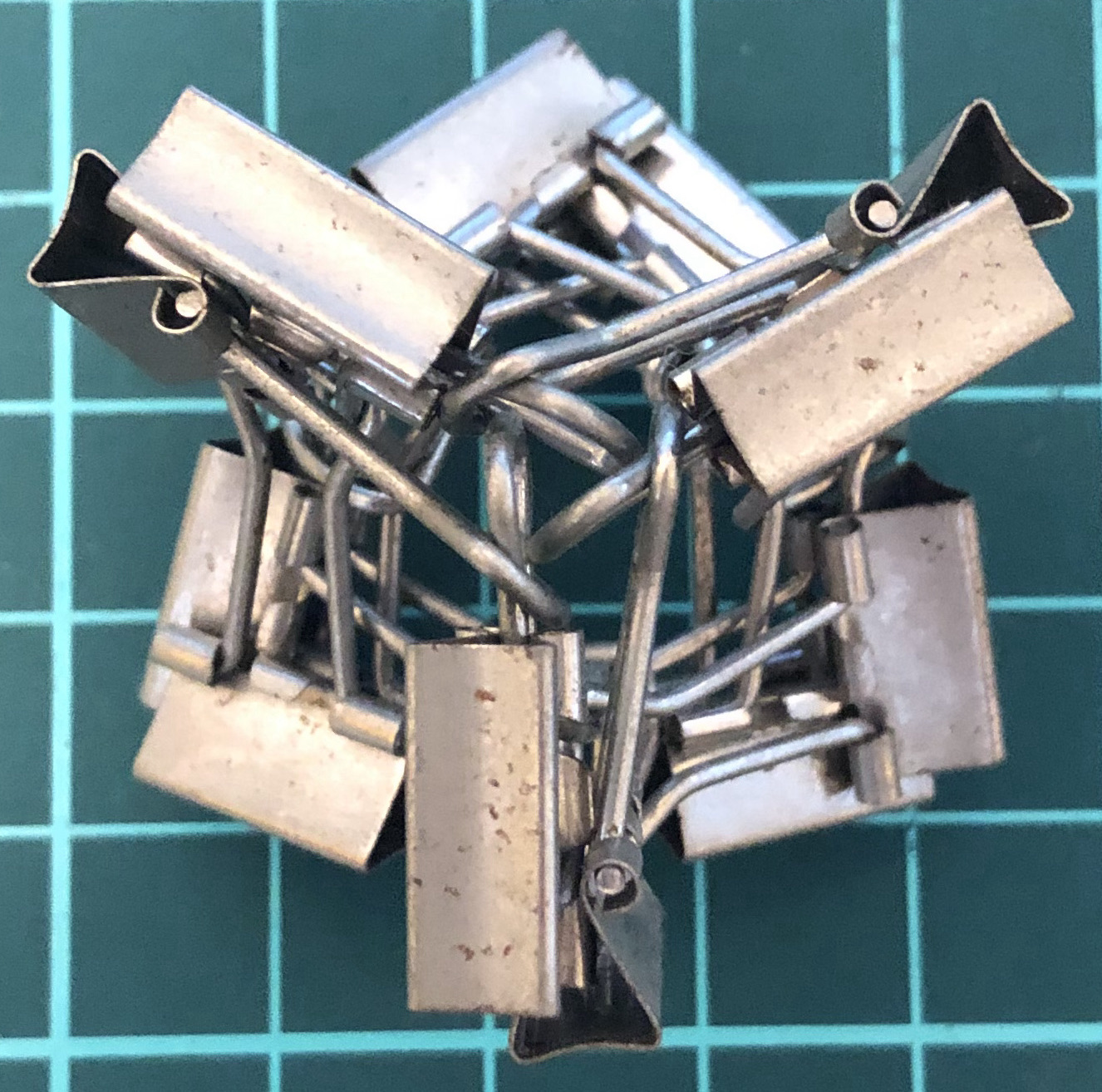↑ # Clips = 12
↑ Base = tetrahedron
↑ Vertex config = 3.3.3
↑ Symmetry = tetrahedron’s rotations = $A_4$ of order 12
↑ Dual = itself

#### L24-C↑ # Clips = 24
↑ Base = cube
↑ Vertex config = 4.4.4
↑ Symmetry = cube’s rotations = $S_4$ of order 24
↑ Dual = L24-O

#### L24-O↑ # Clips = 24
↑ Base = octahedron
↑ Vertex config = 3.3.3.3
↑ Symmetry = cube’s rotations = $S_4$ of order 24
↑ Dual = L24-C

#### L60-D↑ # Clips = 60
↑ Base = dodecahedron
↑ Vertex config = 5.5.5
↑ Symmetry = dodecahedron’s rotations = $A_5$ of order 60
↑ Dual = L60-I

#### L60-I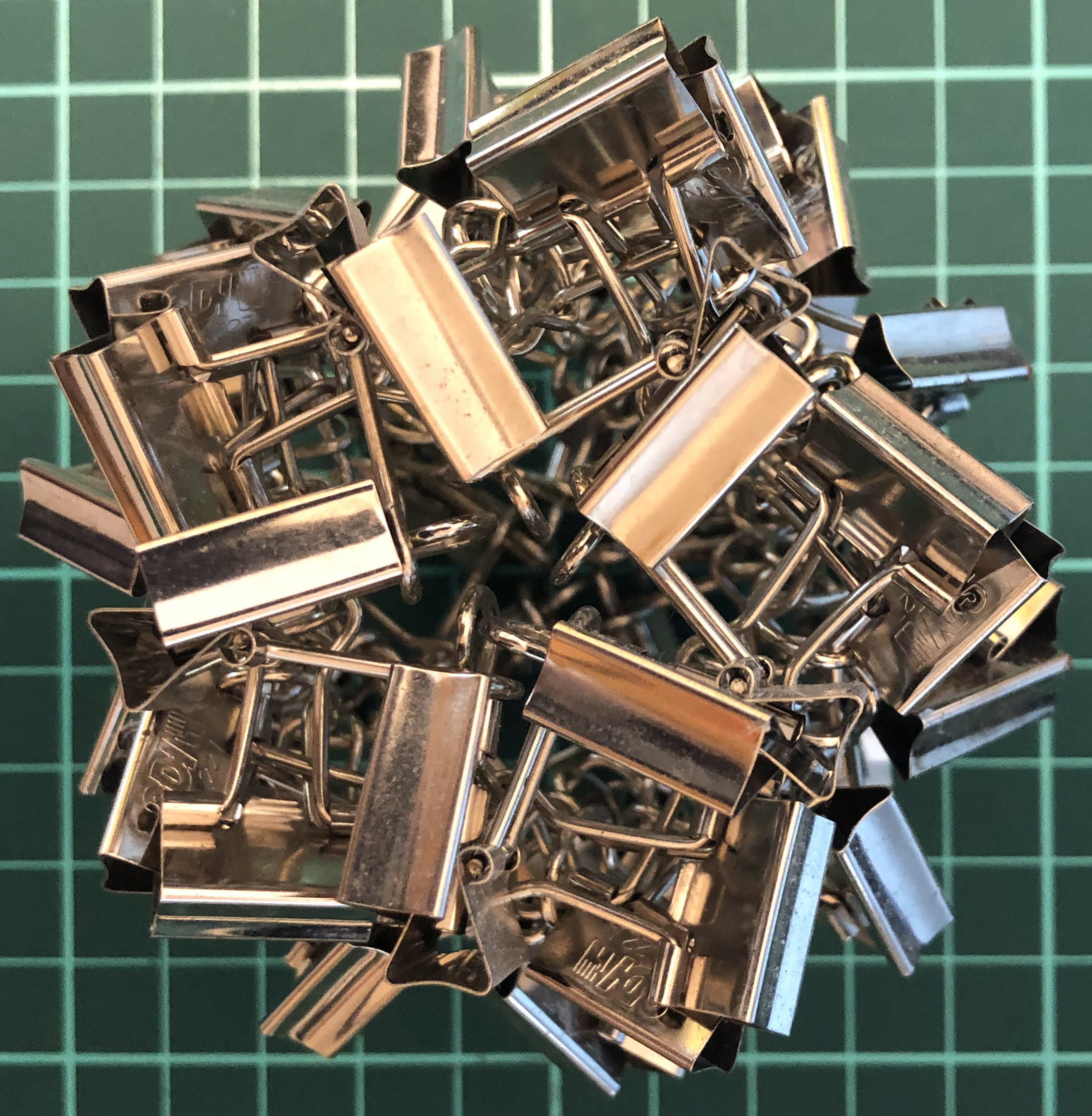↑ # Clips = 60
↑ Base = icosahedron
↑ Vertex config = 3.3.3.3.3
↑ Symmetry = dodecahedron’s rotations = $A_5$ of order 60
↑ Dual = L60-D

#### L36-tT↑ # Clips = 36
↑ Base = truncated tetrahedron
↑ Vertex config = 3.6.6
↑ Symmetry = tetrahedron’s rotations = $A_4$ of order 12

#### L48-aC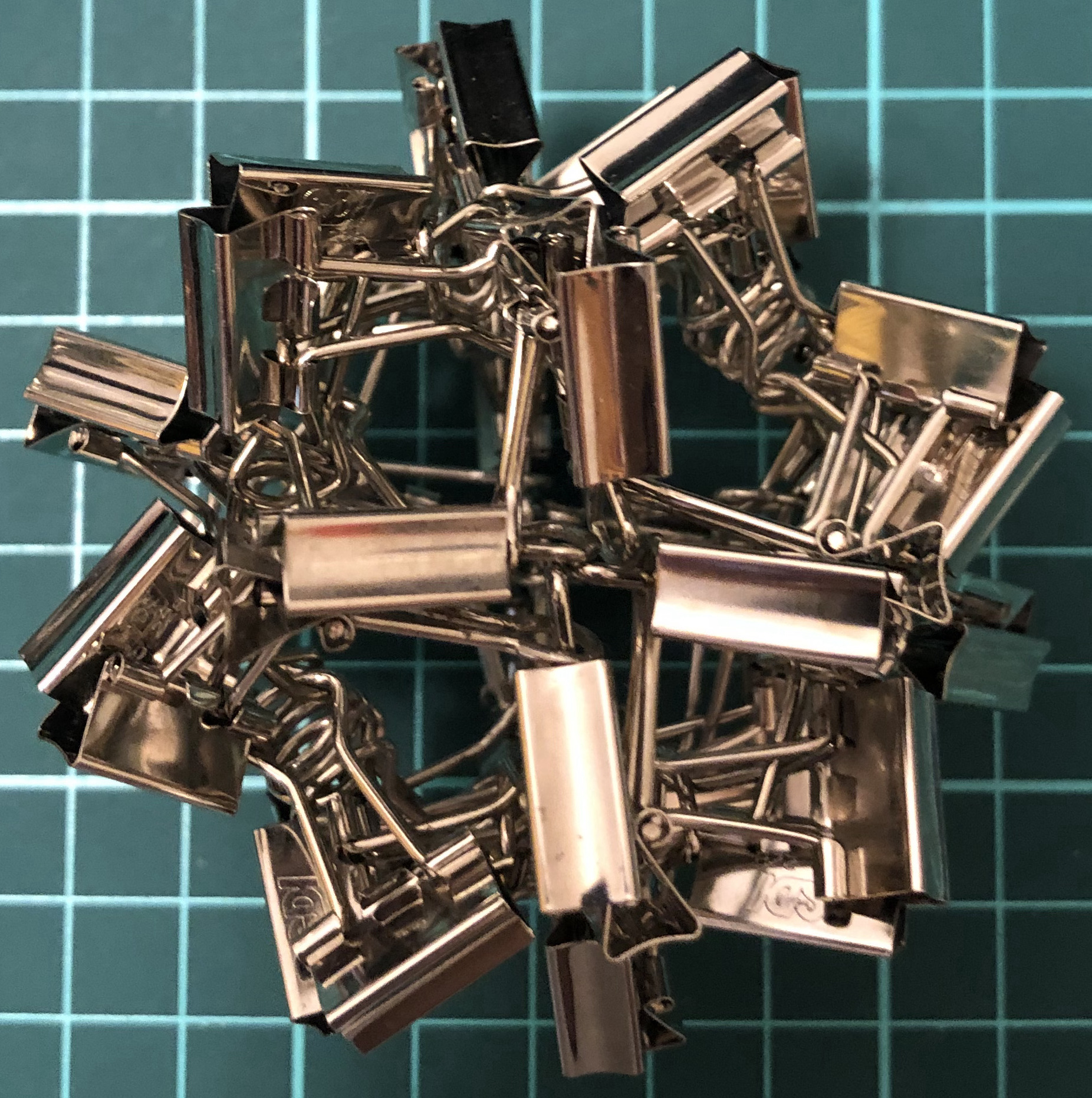↑ # Clips = 48
↑ Base = cuboctahedron
↑ Vertex config = 3.4.3.4
↑ Symmetry = cube’s rotations = $S_4$ of order 24

### I-series, Platonic

Two clips = one I-edge = one edge.

#### I12-T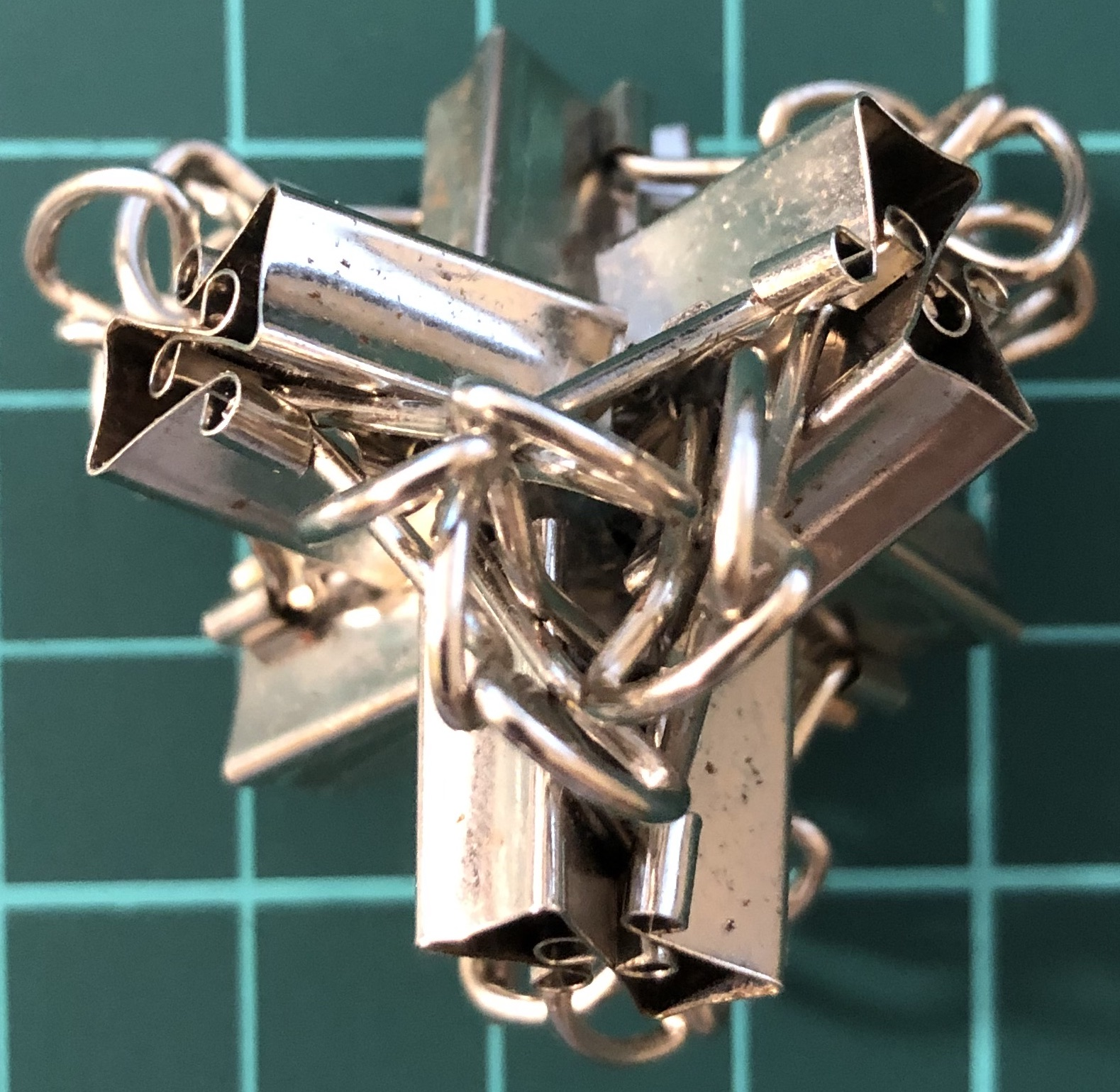↑ # Clips = 12
↑ Base = tetrahedron
↑ Vertex config = 3.3.3
↑ Symmetry = tetrahedron’s rotations = $A_4$ of order 12
↑ Dual = itself

#### I24-C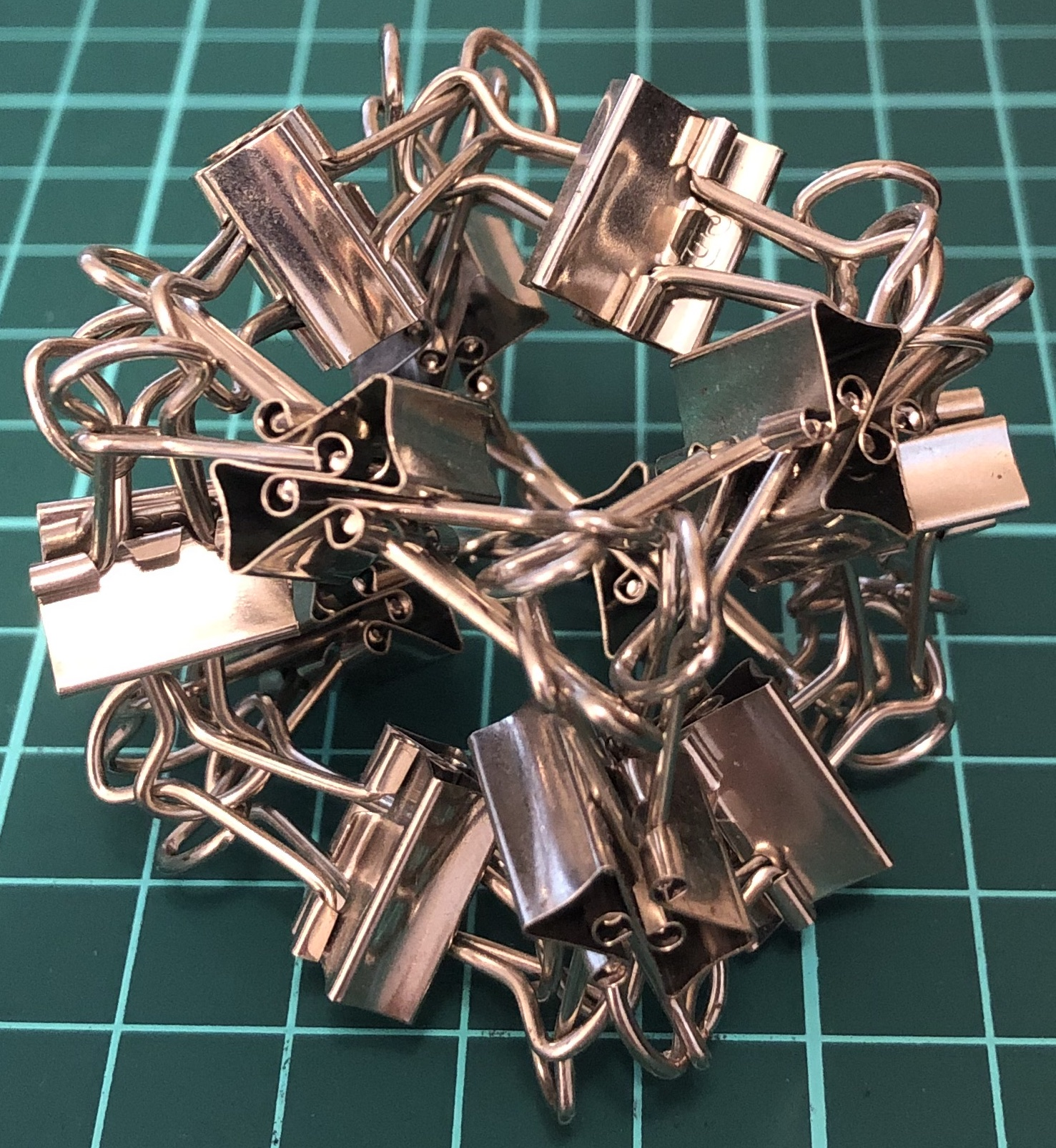↑ # Clips = 24
↑ base = cube
↑ Vertex config = 4.4.4
↑ Symmetry = cube’s rotations = $S_4$ of order 24
↑ dual = I24-O

#### I24-O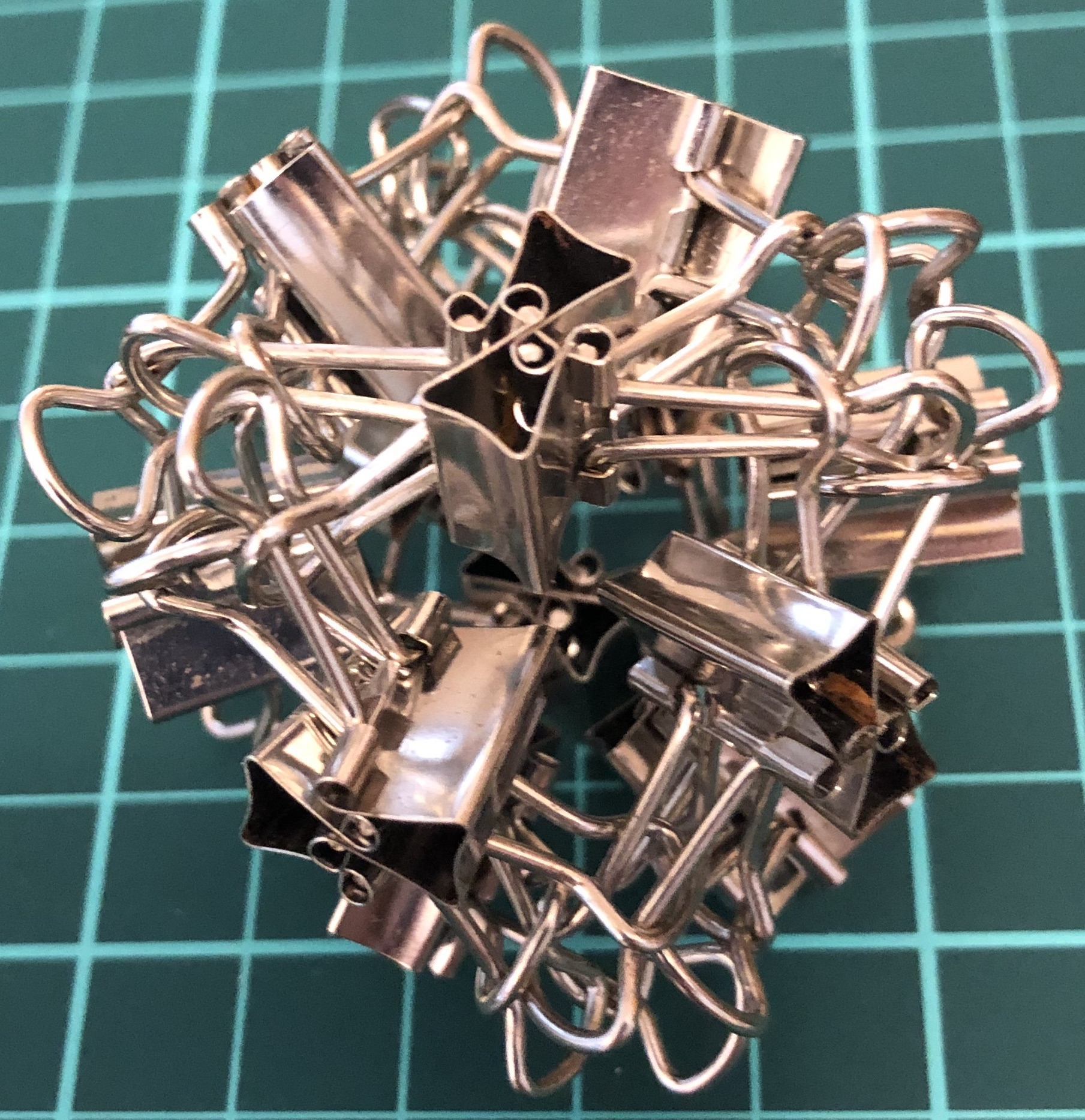↑ # Clips = 24
↑ Base = octahedron
↑ Vertex config = 3.3.3.3
↑ Symmetry = cube’s rotations = $S_4$ of order 24
↑ Dual = I24-C

#### I60-D↑ # Clips = 30
↑ Vertex config = 5.5.5
↑ Base = dodecahedron
↑ Symmetry = dodecahedron’s rotations = $A_5$ of order 60
↑ Dual = I60-I

#### I60-I↑ # Clips = 30
↑ Base = icosahedron
↑ Vertex config = 3.3.3.3.3
↑ Symmetry = dodecahedron’s rotations = $A_5$ of order 60
↑ dual = I60-D

### I-series, Archimedean

#### I36-tT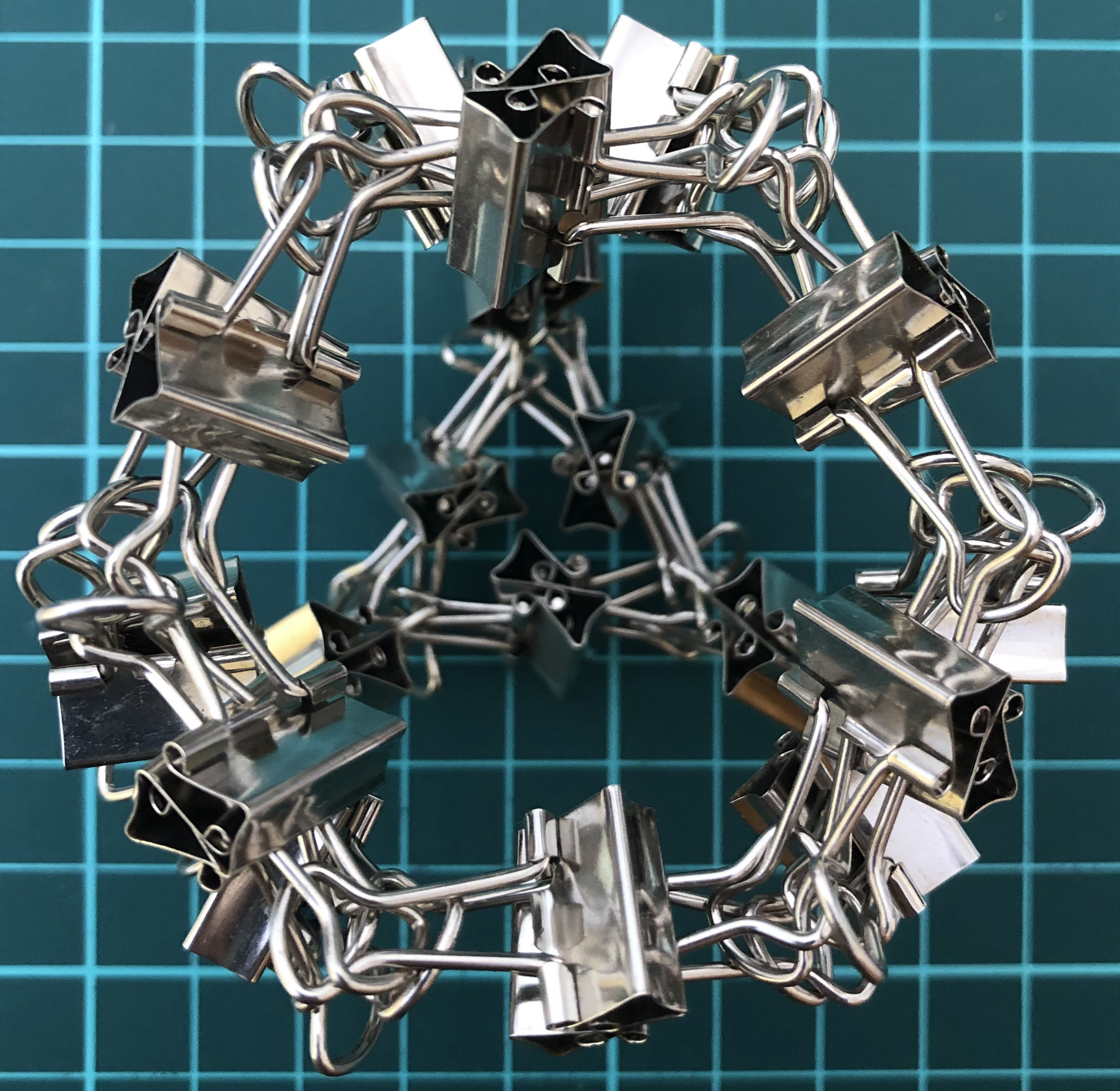↑ # Clips = 36
↑ Base = truncated tetrahedron
↑ Vertex config = 3.6.6
↑ Symmetry = tetrahedron’s rotations = $A_4$ of order 12
↑ Dual = I36-kT

#### I48-aC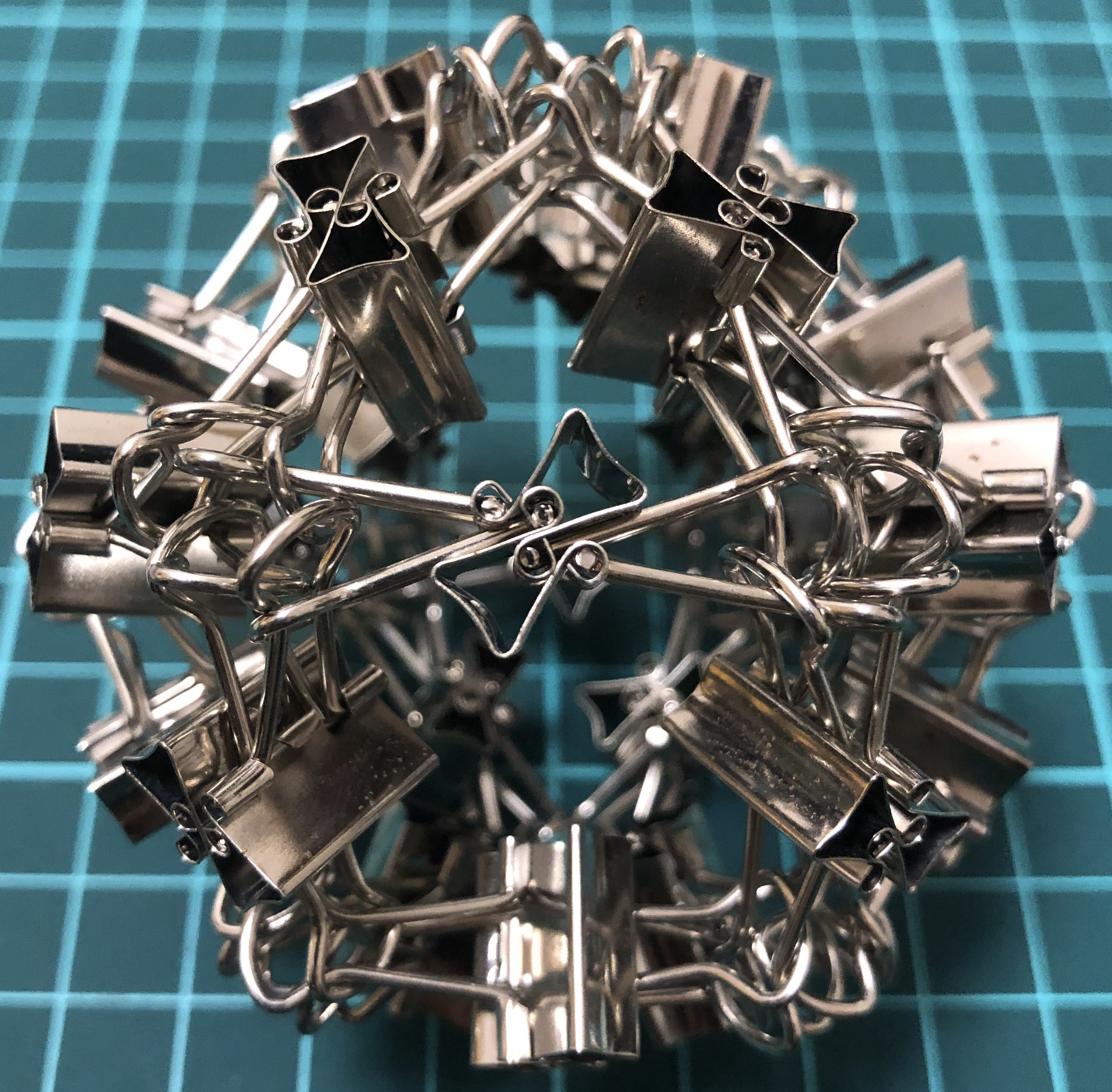↑ # Clips = 48
↑ Base = cuboctahedron
↑ Vertex config = 3.4.3.4
↑ Symmetry = cube’s rotations = $S_4$ of order 24
↑ Dual = I48-jC

#### I72-tC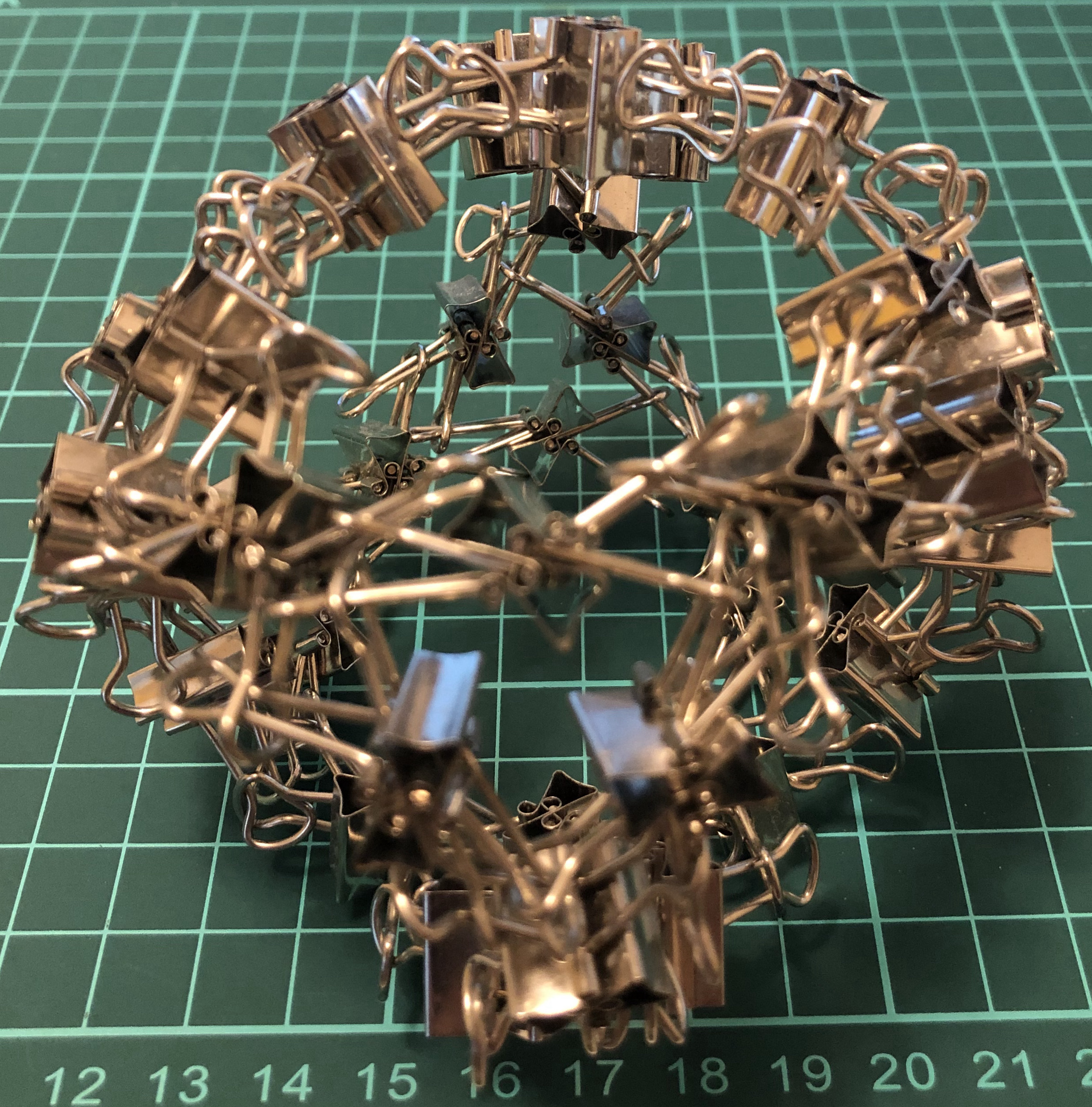↑ # Clips = 72
↑ Base = truncated cube
↑ Vertex config = 3.8.8
↑ Symmetry = cube’s rotations = $S_4$ of order 24
↑ (Dual = triakis octahedron)

#### I72-tO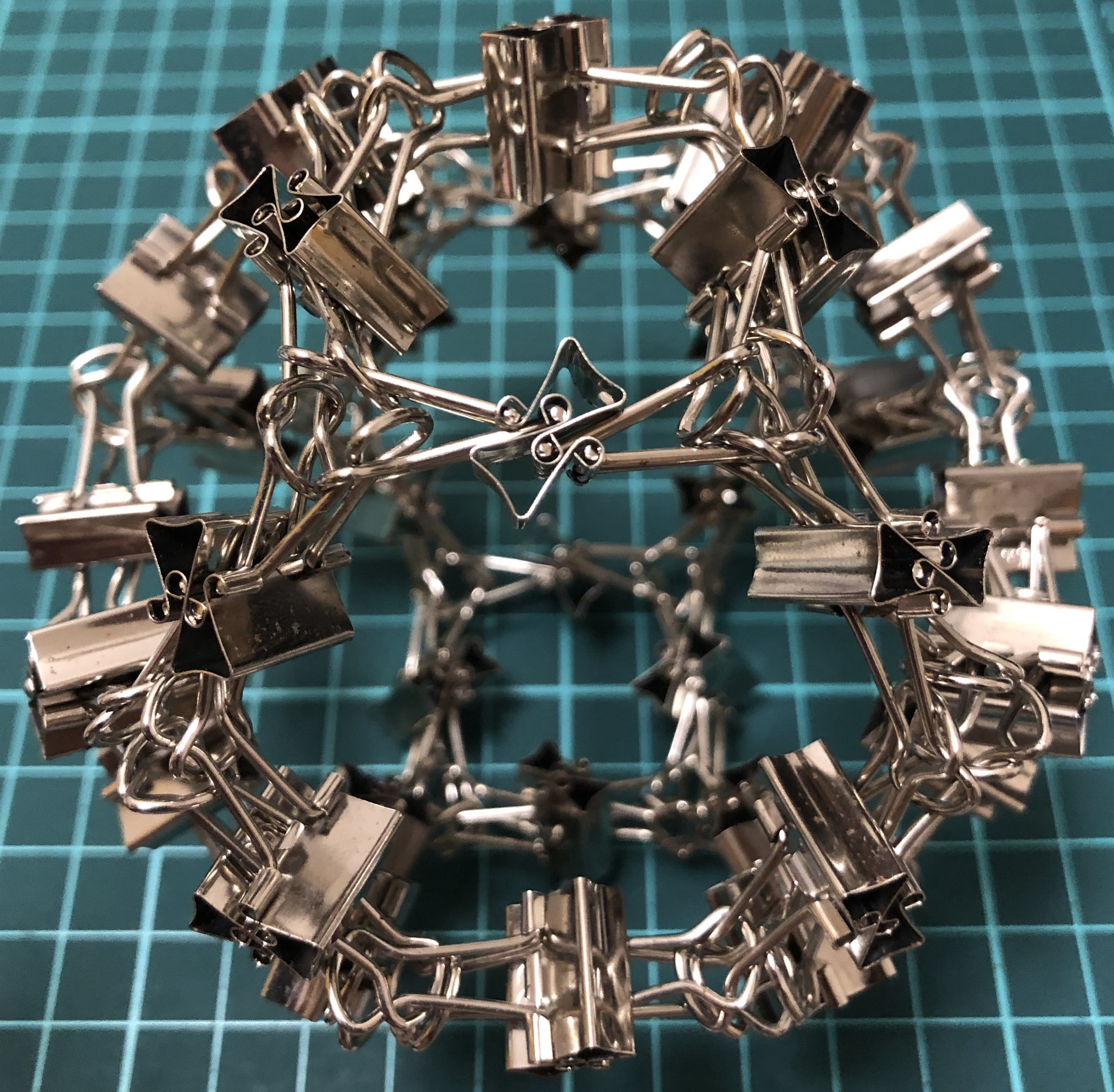↑ # Clips = 72
↑ Base = truncated octahedron
↑ Vertex config = 4.6.6
↑ Symmetry = cube’s rotations = $S_4$ of order 24
↑ (Dual = tetrakis hexahedron)

#### I96-aaC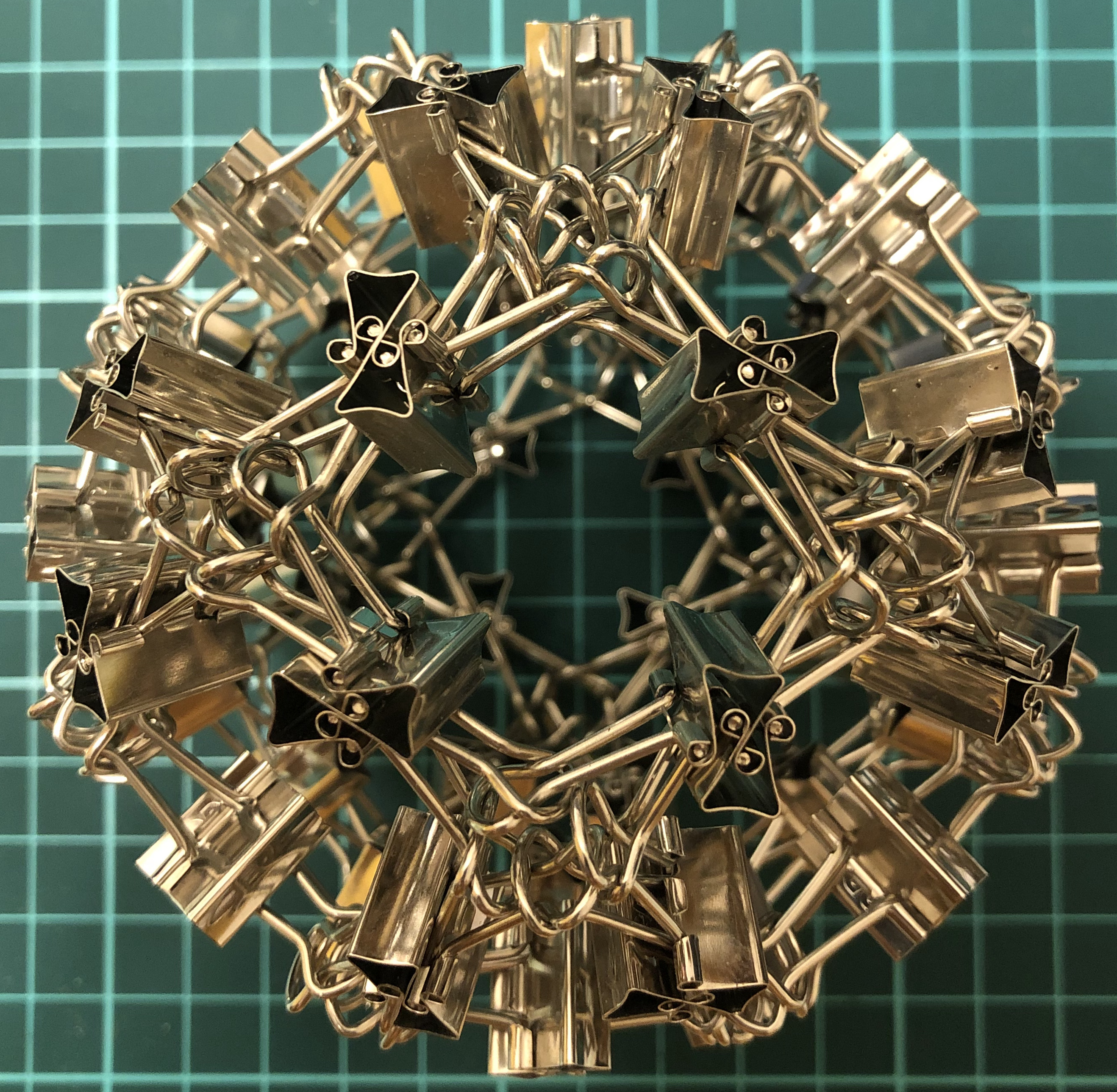↑ # Clips = 96
↑ Base = rhombicuboctahedron
↑ Vertex config = 3.4.4.4
↑ Symmetry = cube’s rotations = $S_4$ of order 24
↑ (Dual = deltoidal icositetrahedron)

#### I120-sC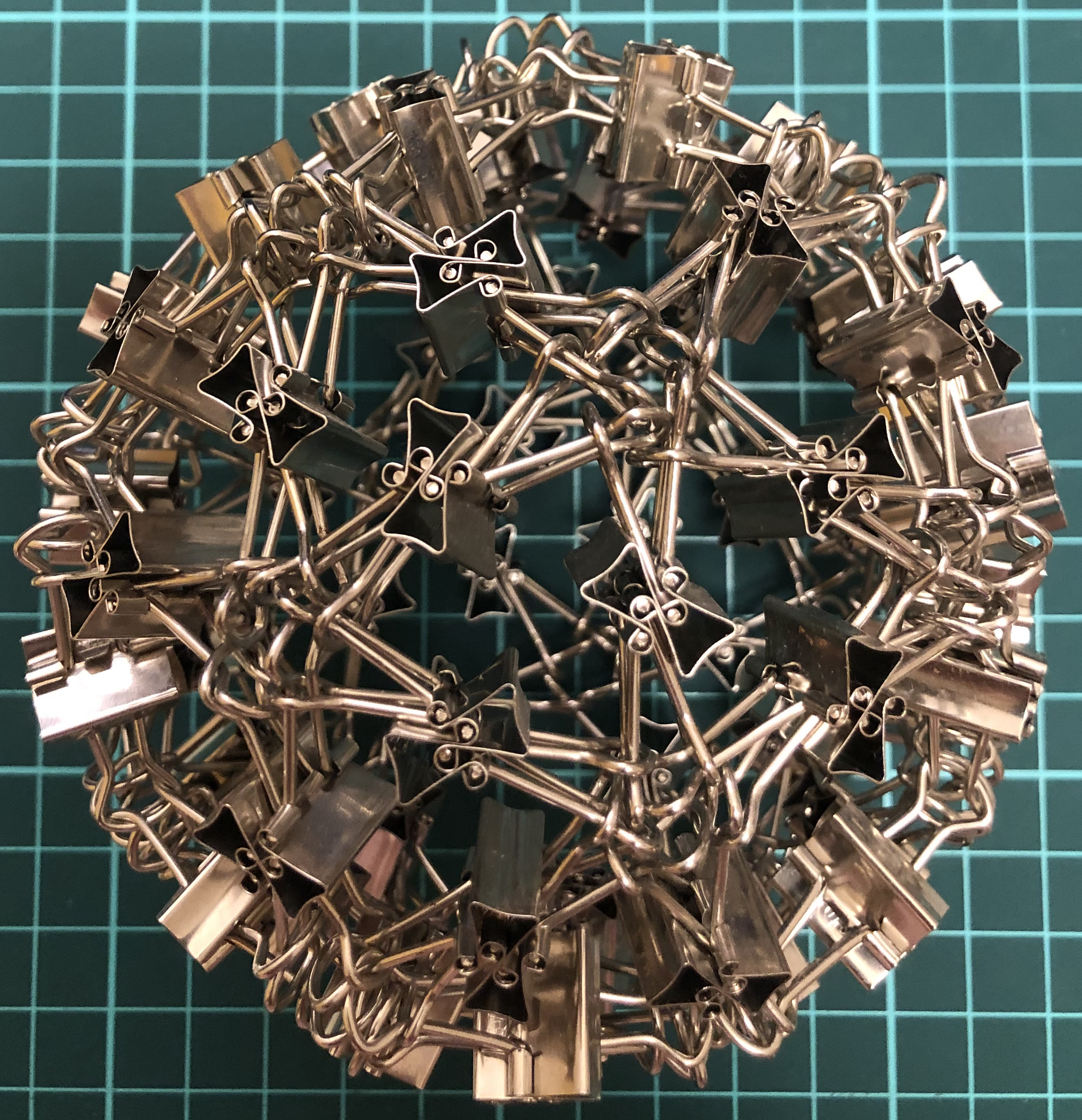↑ # Clips = 120
↑ Base = snub cube
↑ Vertex config = 3.3.3.3.4
↑ Symmetry = cube’s rotations = $S_4$ of order 24
↑ (Dual = pentagonal icositetrahedron)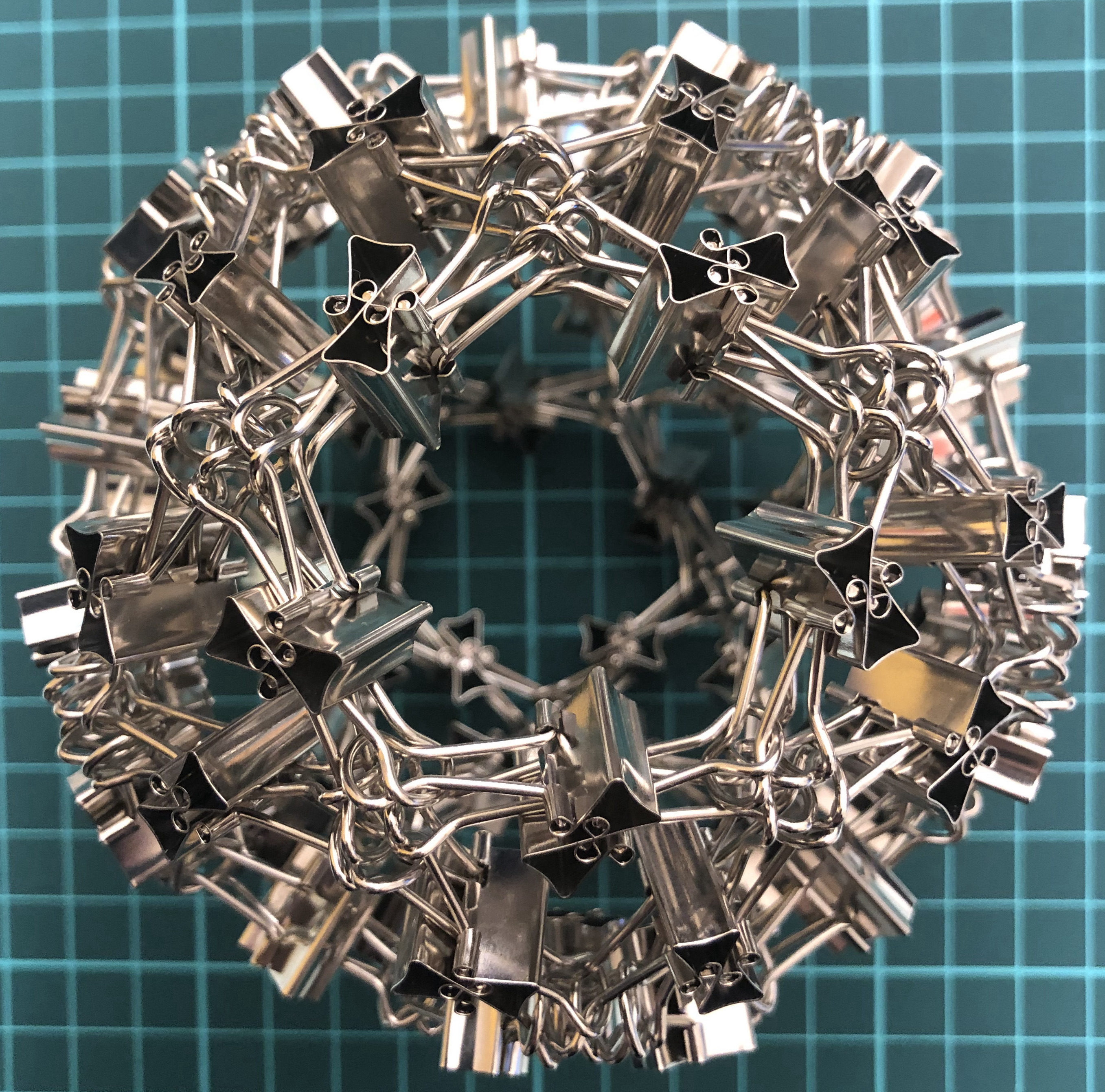↑ # Clips = 120
↑ Base = icosidodecahedron
↑ Vertex config = 3.5.3.5
↑ Symmetry = dodecahedron’s rotations = $A_5$ of order 60
↑ Dual = I120-jD

#### I180-tI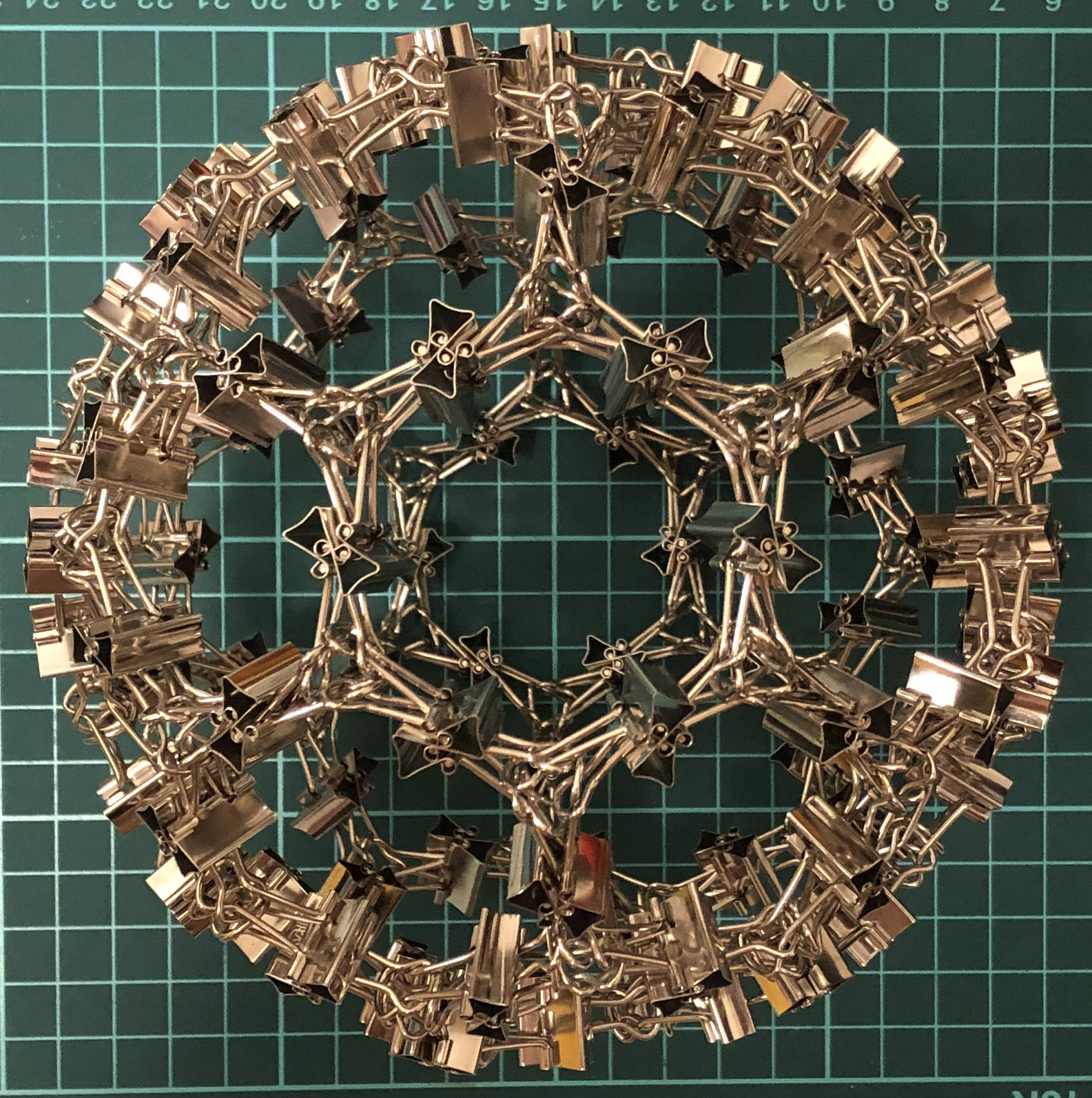↑ # Clips = 180
↑ Base = truncated icosahedron
↑ Vertex config = 5.5.6
↑ Symmetry = dodecahedron’s rotations = $A_5$ of order 60
↑ Dual = I180-kD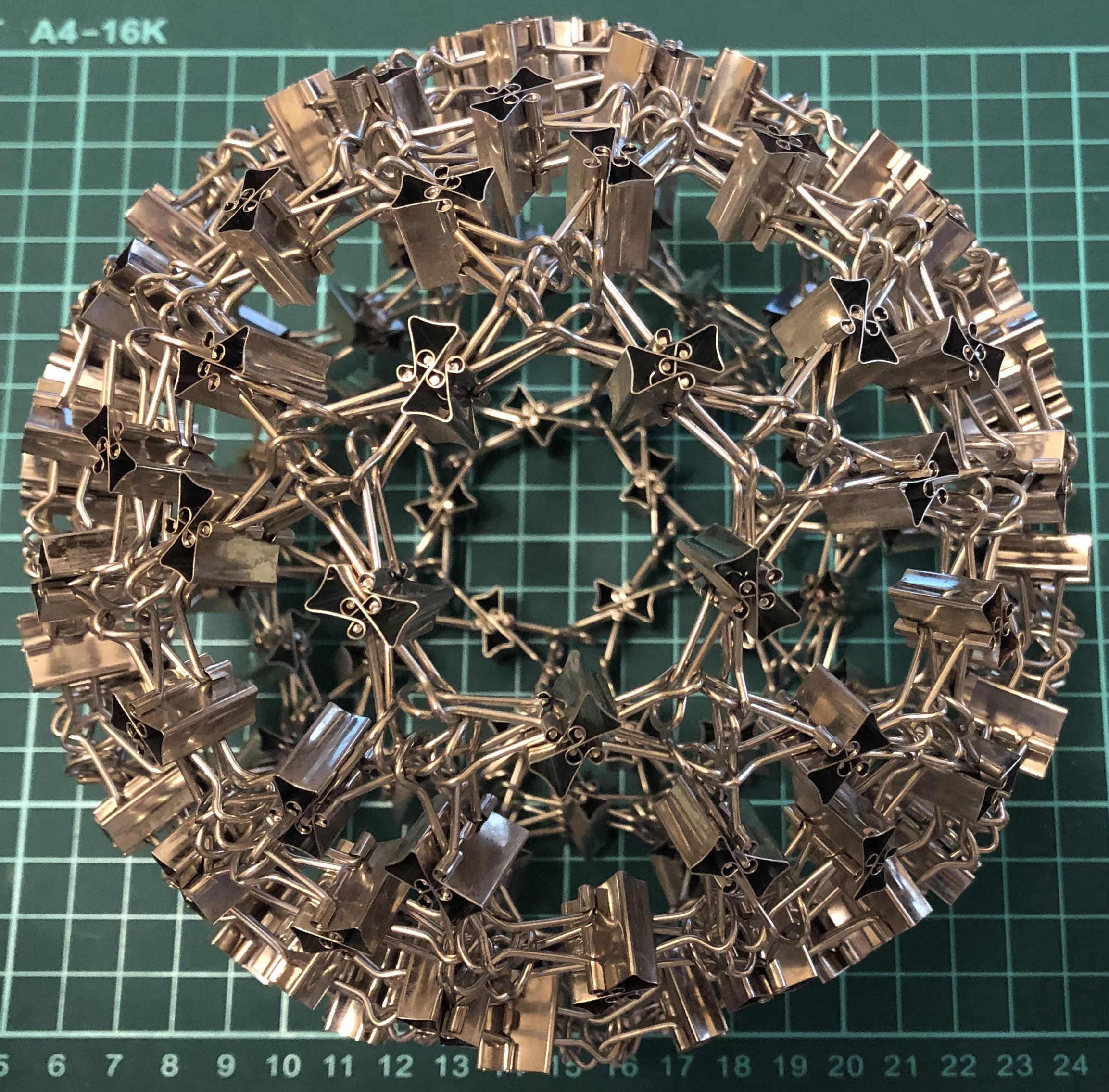↑ # Clips = 240
↑ Base = rhombicosidodecahedron
↑ Vertex config = 3.4.5.4
↑ Symmetry = dodecahedron’s rotations = $A_5$ of order 60

#### I300-sD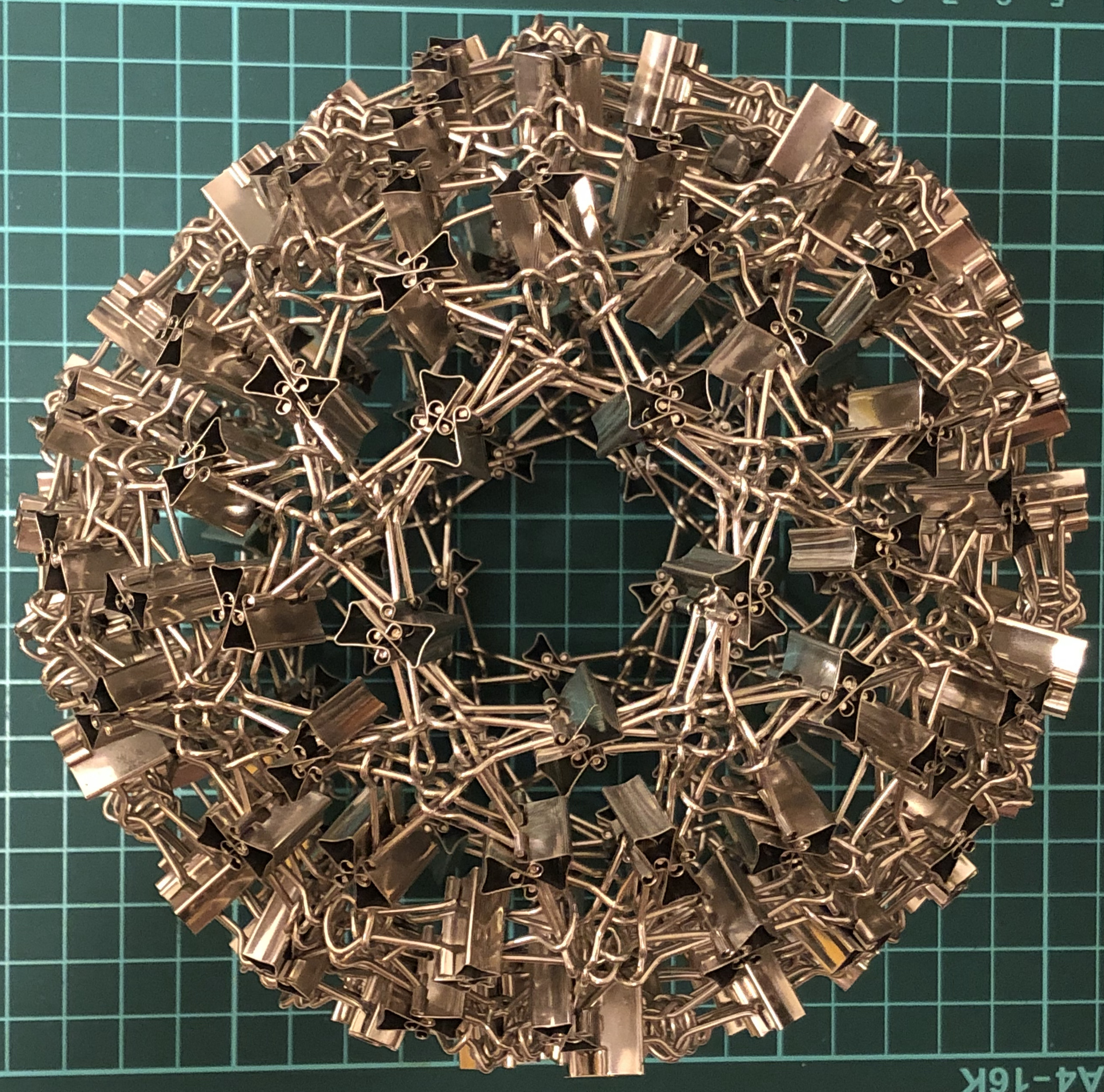↑ # Clips = 300
↑ Base = snub dodecahedron
↑ Face config = 3.3.3.3.5
↑ Symmetry = dodecahedron’s rotations = $A_5$ of order 60
↑ (Dual = pentagonal hexecontahedron)

### I-series, Catalan

#### I36-kT↑ # Clips = 36
↑ Face config = 3.6.6
↑ Base = triakis tetrahedron
↑ Symmetry = tetrahedron’s rotations = $A_4$ of order 12
↑ Dual = I36-tT

#### I48-jC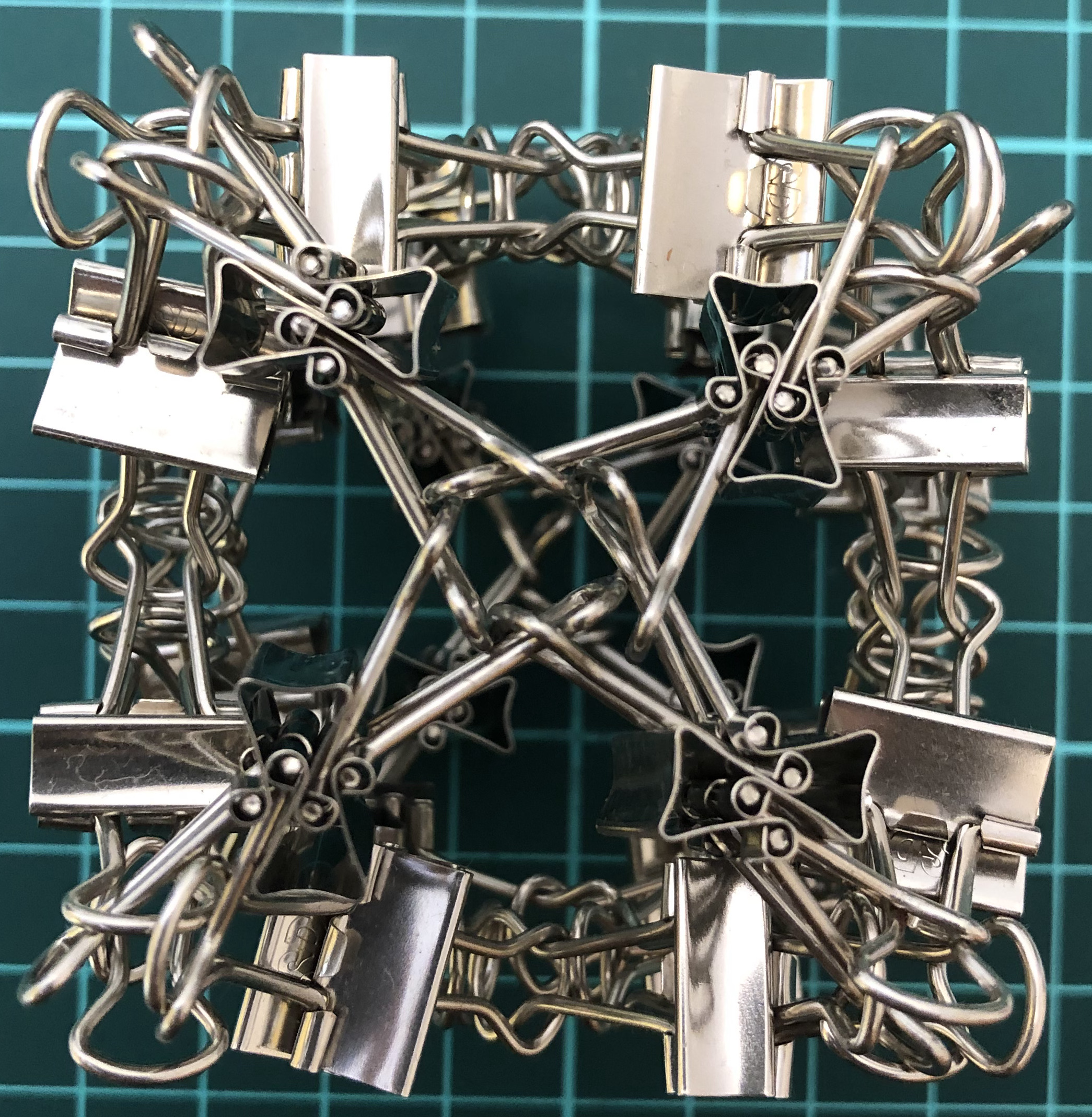↑ # Clips = 48
↑ Base = rhombic dodecahedron
↑ Face config = 3.4.3.4
↑ Symmetry = cube’s rotations = $S_4$ of order 24
↑ Dual = I48-aC

#### I72-kC↑ # Clips = 72
↑ Base = tetrakis hexahedron
↑ Face config = 4.6.6
↑ Symmetry = cube’s rotations = $S_4$ of order 24
↑ Dual = I72-tO

#### I120-jD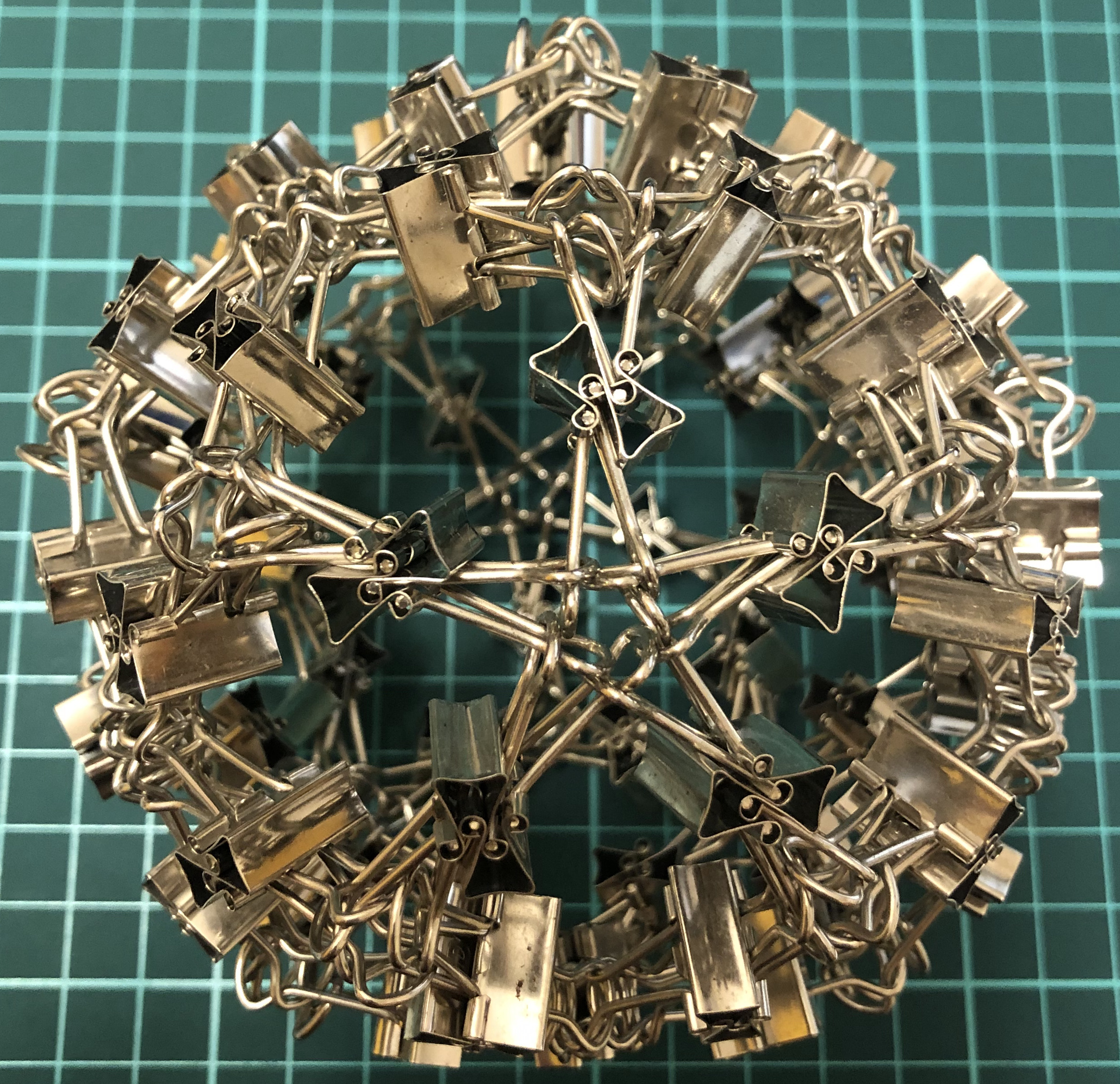↑ # Clips = 120
↑ Base = rhombic triacontahedron
↑ Face config = 3.5.3.5
↑ Symmetry = dodecahedron’s rotations = $A_5$ of order 60

#### I180-kD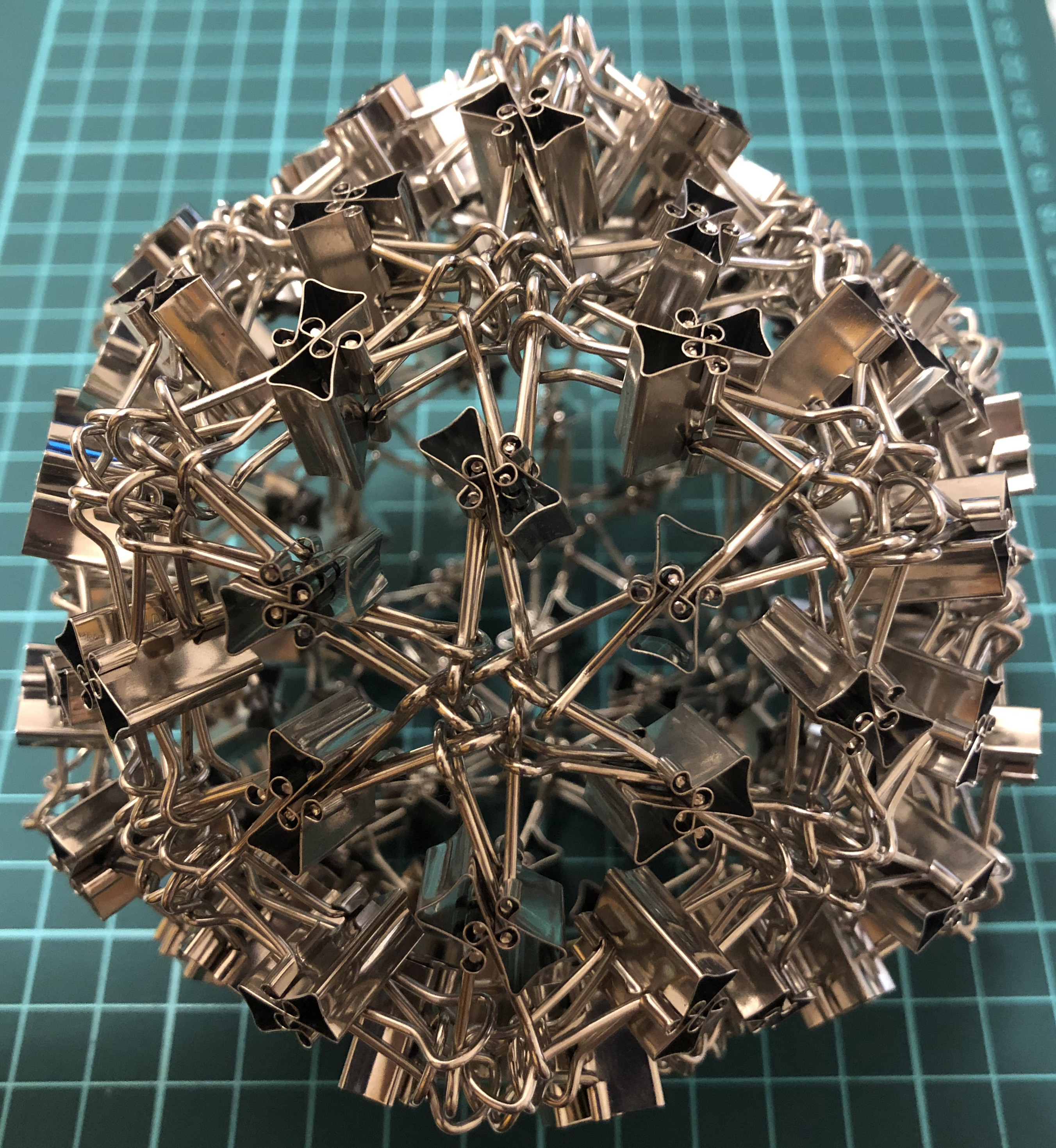↑ # Clips = 120
↑ Base = pentakis dodecahedron
↑ Face config = 5.6.6
↑ Symmetry = dodecahedron’s rotations = $A_5$ of order 60
↑ Dual = I180-tI

### I-series, Fullerene

#### I240-cD↑ # Clips = 240
↑ Base = chamfered dodecahedron
↑ Each dodecahedron vertex = 4 new vertices
↑ Symmetry = dodecahedron’s rotations = $A_5$ of order 60
↑ Dual = I240-uI

#### I240-uI↑ # Clips = 240
↑ Base = pentakis icosidodecahedron aka C80
↑ Each icosahedron face = 4 small triangles
↑ Symmetry = dodecahedron’s rotations = $A_5$ of order 60
↑ Dual = I240-cD

### W-series

#### W12-T↑ # Clips = 12
↑ Base = tetrahedron
↑ Vertex config = 3.3.3
↑ Symmetry = tetrahedron’s rotations = $A_4$ of order 12
↑ Dual = itself

#### W24-C↑ # Clips = 24
↑ base = cube
↑ Vertex config = 4.4.4
↑ Symmetry = cube’s rotations = $S_4$ of order 24
↑ dual = W24-O

#### W24-O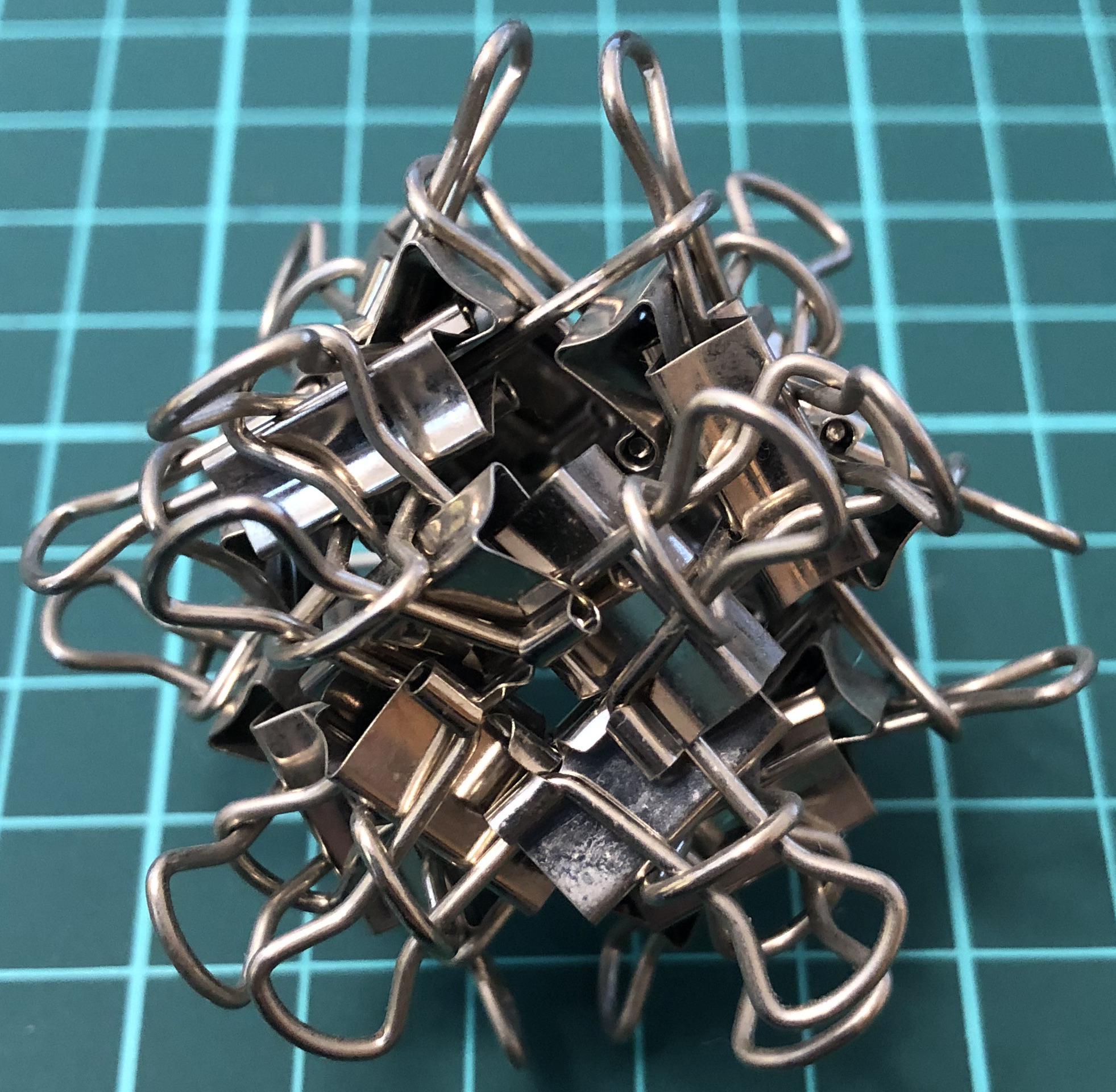↑ # Clips = 24
↑ base = octahedron
↑ Vertex config = 3.3.3.3
↑ Symmetry = cube’s rotations = $S_4$ of order 24
↑ dual = W24-C

#### W60-D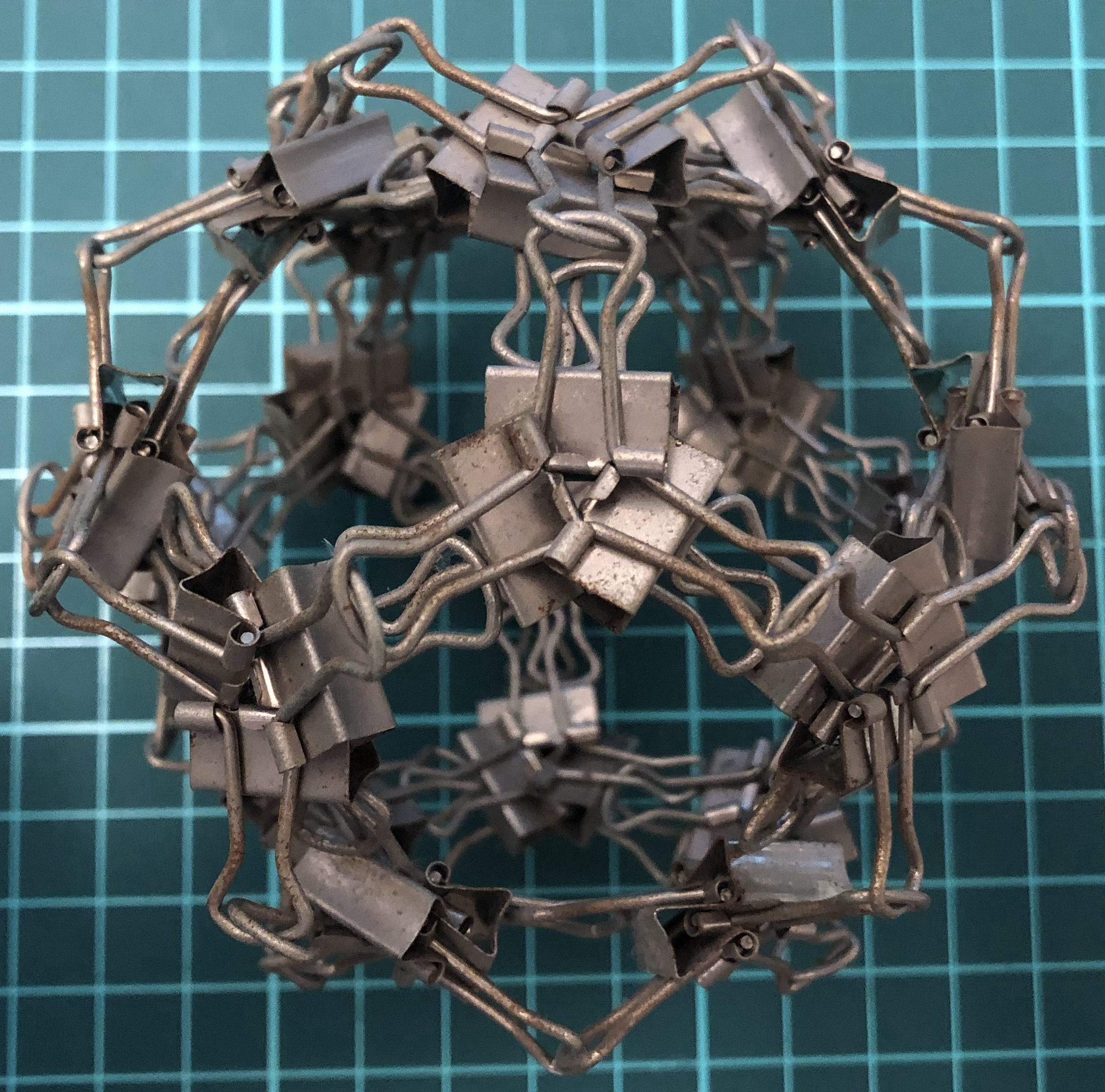↑ # Clips = 60
↑ Base = dodecahedron
↑ Vertex config = 5.5.5
↑ Symmetry = dodecahedron’s rotations = $A_5$ of order 60
↑ Dual = W60-I

#### W60-I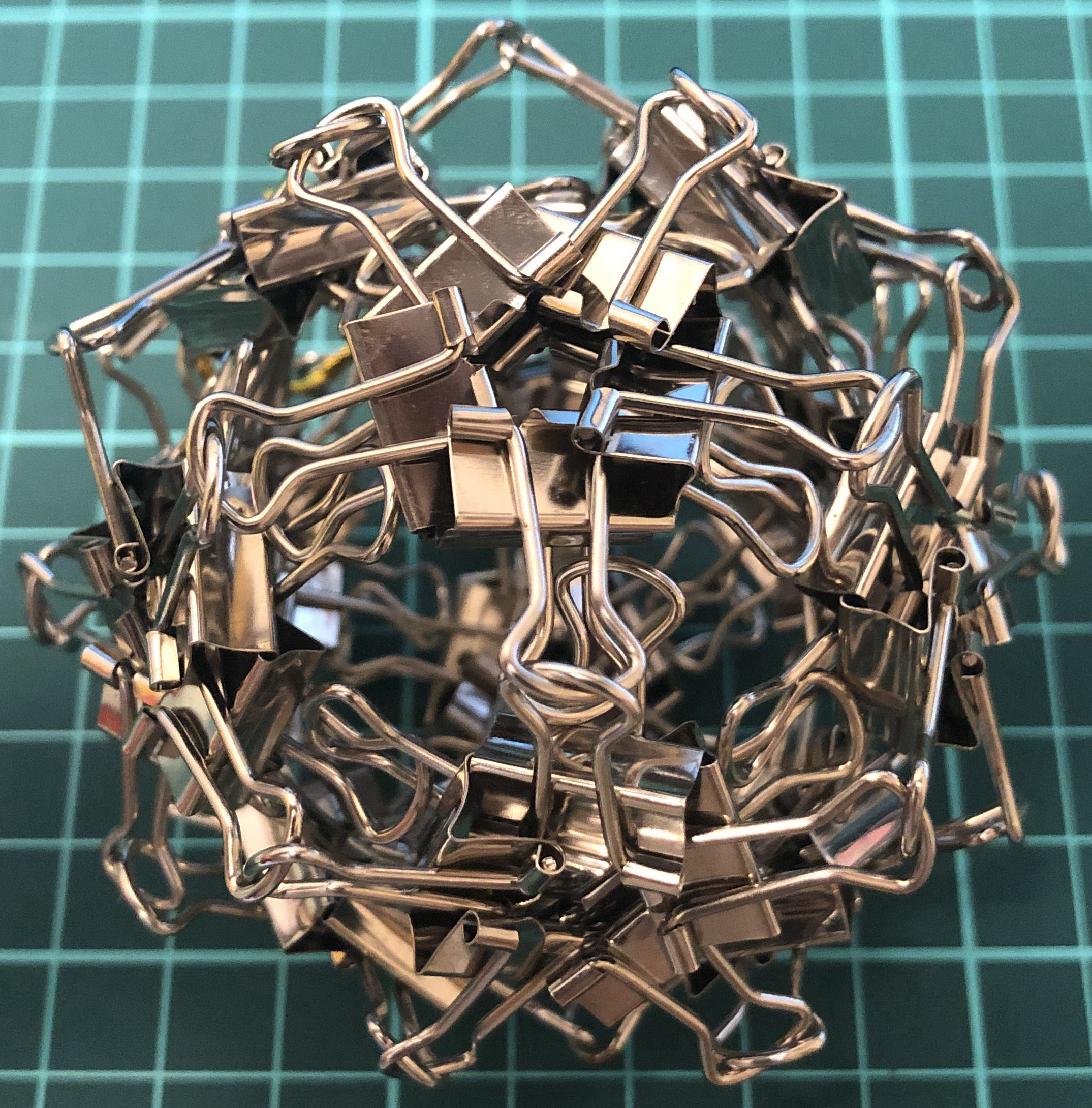↑ # Clips = 60
↑ Base = icosahedron
↑ Vertex config = 3.3.3.3.3
↑ Symmetry = dodecahedron’s rotations = $A_5$ of order 60
↑ Dual = W60-D

#### W36-tT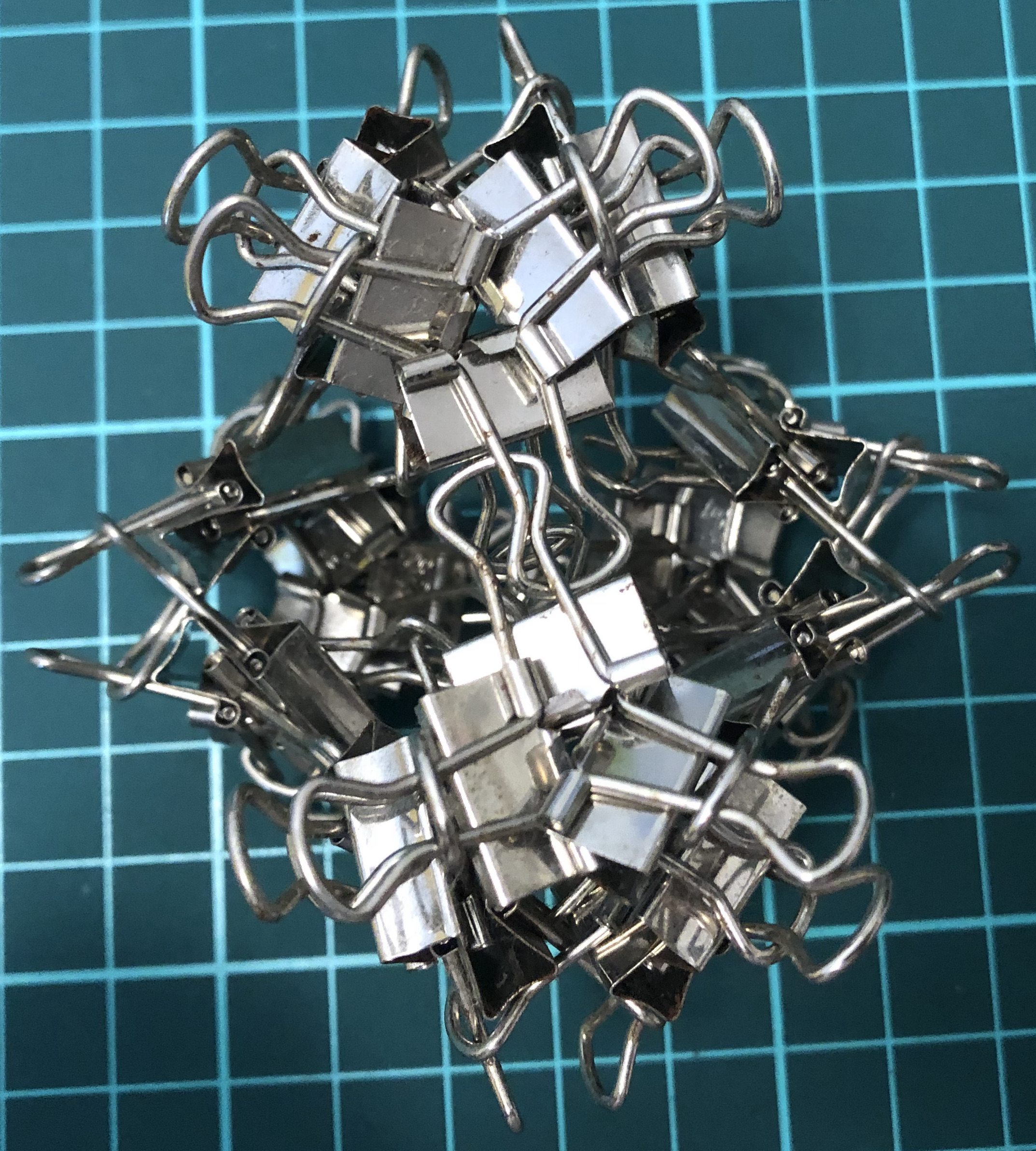↑ # Clips = 36
↑ Base = truncated tetrahedron
↑ Vertex config = 3.6.6
↑ Symmetry = tetrahedron’s rotations = $A_4$ of order 12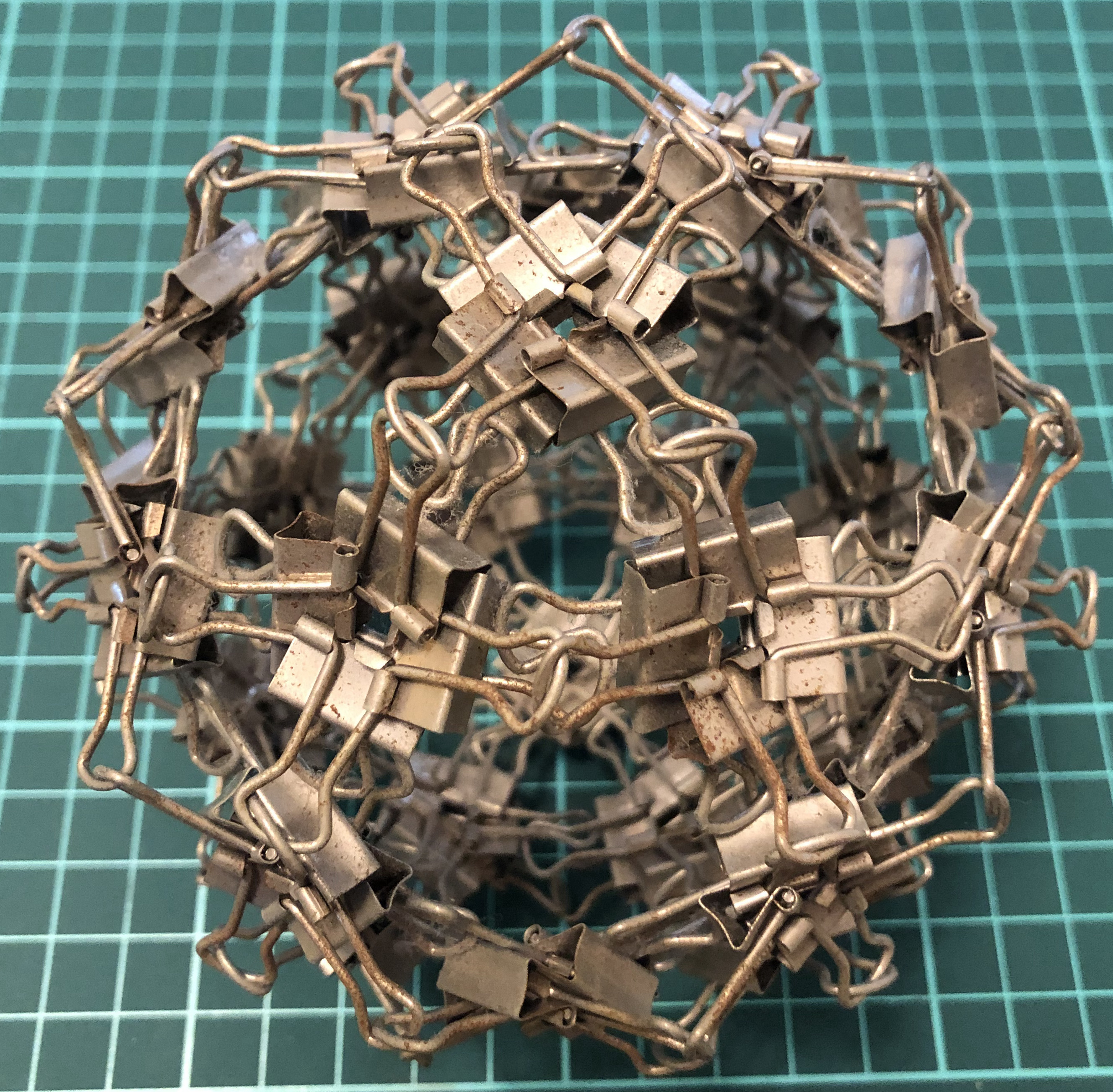↑ # Clips = 120
↑ Base = icosidodecahedron
↑ Vertex config = 3.5.3.5
↑ Symmetry = dodecahedron’s rotations = $A_5$ of order 60